# Deep Dreams of a CNN trained on MNIST data – I – a first approach based on one selected map of a convolutional layer

It is fun to play around with Convolutional Neural Networks [CNNs] on the level of an dedicated amateur. One of the reasons is the possibility to visualize the output of elementary building blocks of this class of AI networks. The resulting images help to understand CNN algorithms in an entertaining way – at least in my opinion. The required effort is in addition relatively limited: You must be willing to invest a bit of time into programming, but on a quite modest level of difficulty. And you can often find many basic experiments which are in within the reach of limited PC capabilities.

A special area where the visualization of CNN guided processes is the main objective is the field of “Deep Dreams“. Anyone studying AI methods sooner or later stumbles across the somewhat psychedelic, but none the less spectacular images which Google presented in 2016 as a side branch of their CNN research. Today, you can download DeepDream generators from GitHub.

When I read a bit more about “DeepDream” experiments, I quickly learned that people use quite advanced CNN architectures, like Google’s Inception CNNs, and apply them to high resolution images (see e.g. the Book of F. Chollet on “Deep Learning with Keras and Python” and ai.googleblog.com, 2015, inceptionism-going-deeper-into-neural). Even if you pick up an already trained version of an Inception CNN, you need some decent GPU power to do your own experiments. Another questionable point for an interested amateur is: What does one actually learn from applying “generators”, which others have programmed, and what from just following a “user guide” without understanding what a DeepDream SW actually does? Probably not much, even if you produce stunning images after some time…

So, I asked myself: Can one study basic methods of the DeepDream technology with self programmed tools and a simple dataset? Could one create a “DeepDream” visualization with a rather simply structured CNN trained on MNIST data?
The big advantage of the MNIST data set is that the individual samples are small; and the amount of numerical operations, which a related simple CNN must perform on input images, fits well to the capabilities of PC technology – even if the latter is some years old.

After a first look into DeepDream algorithms, I think: Yes, it should be possible. In a way DeepDream experiments are a natural extension of the visualization of CNN filters and maps which I have already discussed in depth in another article series. Therefore, DeepDream visualizations might even help us to better understand how the internal filter of CNNs work and what “features” are. However, regarding the creation of spectacular images we need to reduce our expectations to a reasonably low level:

A CNN trained on MNIST data works with gray images, low resolution and only simple feature patterns. Therefore, we will never produce such impressive images as published by DeepDream artists or by Google. But, we do have a solid chance to grasp some basic principles and ideas of this side-branch of AI with very simplistic tools.

As always in this blog, I explore a new field step-wise and let you as a reader follow me through the learning process. Throughout most of this new series of articles we will use a CNN created with the help of Keras and filter visualization tools which were developed in another article series of this blog. The CNN has been trained on the MNIST data set already.

In this first post we are going to pick just a single selected feature or response map of a deep CNN layer and let it “dream” upon a down-scaled image of roses. Well, “dream“, as a matter of fact, is a misleading expression; but this is true for the whole DeepDream
business – as we shall see. A CNN does not dream; “DeepDream” creation is more to be seen as an artistic discipline using algorithmic image enhancement.

The input image which we shall feed into our CNN today is shown below:

As our CNN works on a resolution level of 28×28 pixels, only, the “dreaming” will occur in a coarse way, very comparable to hallucinations on the blurred vision level of a short-sighted, myopic man. More precisely: Of a disturbed myopic man who works the whole day with images of digits and lets this poor experience enter and manipulate his dreamy visions of nicer things :-).

Actually, the setup for this article’s experiment was a bit funny: I got the input picture of roses from my wife, who is very much interested in art and likes flowers. I am myopic and in my soul still a theoretical physicist, who is much more attracted by numbers and patterns than by roses – if we disregard the interesting fractal nature of rose blossoms for a second :-).

# What do DeepDreams based on single maps of trained MNIST CNNs produce?

To rouse your interest a bit or to disappoint you from the start, I show you a typical result of today’s exercise: “Dreams” or “hallucinations” based on MNIST and a selected single map of a deep convolutional CNN layer produce gray scale images with ghost-like “apparitions”.

When these images appeared on my computer screen, I thought: This is fun, indeed! But my wife just laughed – and said “physicists” with a known undertone and something about “boys and toys” …. I hope this will not stop you from reading further. Later articles will, hopefully, produce more “advanced” hallucinations. But as I said: It all depends on your expectations.

But, lets focus: How did I create the simple “dream” displayed above?

# Requirements – a CNN and analysis and visualization tools described in another article series of this blog

I shall use results and methods, which I have already explained in another article series. You need a basic understanding of how a CNN works, what convolutional layers, kernel based filters and cost functions are, how we can build simple CNNs with the help of Keras, … – otherwise you will be lost from the beginning.
A simple CNN for the MNIST datasets – I – CNN basics
We also need a CNN, already trained on the MNIST data. I have shown how to build and train a very simple, yet suitable CNN with the help of Keras and Python; see e.g.:
A simple CNN for the MNIST datasets – II – building the CNN with Keras and a first test
A simple CNN for the MNIST dataset – III – inclusion of a learning-rate scheduler, momentum and a L2-regularizer
code to create input image patterns which trigger response maps or full layers of a CNN optimally. I called such pixel patterns “OIPs”; others call them “features”. I have offered a Python class in the other article series which offers an optimization loop and other methods to work on OIPs and filter visualization.
A simple CNN for the MNIST dataset – XI – Python code for filter visualization and OIP detection

We shall extend this class by further methods throughout our forthcoming work. To develop and run the codes you should have a working Jupyter environment, a virtual Python environment, an IDE like Eclipse with PyDev for building larger code segments and a working Cuda installation for a NVidia graphics card. My 960GTX proved to be fully sufficient for what we are going to do.

# Deep “Dream” – or some funny image manipulation?

As it unfortunately happens so often with AI topics: Also in case of the term “DeepDream” the vocabulary is exaggerated and thoroughly misleading. A simple CNN neither thinks nor “dreams” – it is a software manifestation of the results of an optimization algorithm applied to and trained on selected input data. If applied to new input, it will only detect patterns for which it was optimized before. You could also say:

A CNN is a manifestation of learned prejudices.

CNNs and other types of AI networks filter input according to encoded rules which serve a specific purpose and which reflect the properties of the selected training data set. If you ever used the CNN of my other series on your own hand-written images after a training only on the (US-) MNIST images you will quickly see what I mean. The MNIST dataset reflects an American style of writing digits – a CNN trained on MNIST will fail relatively often when confronted with image samples of digits written by Europeans.

Why do I stress this point at all? Because DeepDreams reveal such kinds of “prejudices” in a visible manner. DeepDream technology extracts and amplifies patterns within images, which fit the trained filters of the involved CNN. F. Chollet correctly describes “DeepDream” as an image manipulation technique which makes use of algorithms for the visualization of CNN filters.

The original algorithmic concept for DeepDreams consists of the following steps:

• Extend your algorithm for CNN filter visualization (= OIP creation) from a specific map to the optimization of the response of complete layers. Meaning: Use the total response of all maps of a layer to define contributions to your cost function. Then mix these contributions in a defined weighted way.
• Take some image of whatever motive you like and prepare 4 or more down-scaled versions of this image, i.e. versions with different levels of size and resolution below the original size and resolution.
• Offer the image with the lowest resolution to the CNN as an input image.
• Loop over all prepared image sizes :
• Apply your algorithm for filter visualization of all maps and layers to the input image – but only for a very limited amount of epochs.
• Upscale the resulting output image (OIP-image) to the next level of higher resolution.
• Add details of the original image with the same resolution to the upscaled OIP-image.
• Offer the resulting image as a new input image to your CNN.

Readers who followed me through my last series on “a simple CNN for MNIST” should already raise their eyebrows: What if the CNN expects a certain fixed size of of the input image? Well, a good question. I’ll come back to it in a second. For the time being, let us say that we will concentrate more on resolution than on an
actual image size.

The above steps make it clear that we manipulate an image multiple times. In a way we transform the image slowly to improve a layer’s response and repeat the process with growing resolution. I.e., we apply pattern detection and amplification on more and more details – in the end using all available large and small scale filters of the CNN in a controlled way without fully eliminating the original contents.

# What to do about the low resolution of MNIST images and the limited capability of a CNN trained on them?

MNIST images have a very low resolution, real images instead a significantly higher one. With our CNN specialized on MNIST input the OIP-creation algorithm only works on (28×28)-images (and with some warnings, maybe, on smaller ones). What to do about it when we work with input images of a size of e.g. 560×560 pixels?

Well, we just work on the given level of resolution! We have three options:

• We can downsize the input image itself or parts of it to the MNIST dimensions – with the help of a bicubic interpolation. Then our OIP-algorithm has the chance to detect OIPs on the coarse scale and to change the downsized image accordingly. Then we can upscale the result again to the original image size – and add details again.
• We can split the input image into tiles of size (28×28) and offer these tiles as input to the CNN.
• We can combine both of the above options.

Its like what a shortsighted human would do: Work with a blurred impression of the full scale image or look at parts of it from a close distance and then reassemble his/her impressions to larger scales.

# A first step – apply only one specific map of a convolutional layer on a down-scaled image version

In this article we have a very limited goal for which we do not have to change our tools, yet:

• Preparation:
• We choose a map.
• We downscale the original image to (28×28) by interpolation, upscale the result again by interpolating again (with loss) and calculate the difference to the original full resolution image (all interpolations done in a bicubic way).
• Loop (4 times or so):
• We apply the OIP-algorithm on the downscaled input image for a fixed amount of epochs
• We upscale the result by bicubic interpolation to the original size.
• We re-add the difference in details.
• We downscale the result again.

With this approach I try to apply some of the elements of the original algorithm – but just on one scale of coarse resolution. I shall discuss the code for realizing the recipe given above with Python and Jupyter in the next article. For today let us look at some of the ghost like apparitions in the dreams for selected maps of the 3rd convolutional layer; see:
A simple CNN for the MNIST dataset – IX – filter visualization at a convolutional layer

# DeepDreams based on selected maps of the 3rd convolutional layer of a CNN trained on MNIST data

With the image sections displayed below I have tried to collect results for different maps which focus on certain areas of the input image (with the exception of the first image section).

The first two images of each row display the detected OIP-patterns on the (28×28) resolution level with pixel values encoded in a (viridis) color-map; the third image in gray scale. The fourth image reveals the dream on the blurry vision level – up-scaled and interpolated to the original image size. You may still detect traces of the original rose blossoms i these images. The last two images of each row display the results
after re-adding details of the original image and an adjustment of the width of the value distribution. The detected and enhanced pattern then turns into a whitey, ghostly shadow.

I have given each section a fancy headline.

I never promised you a rose garden …“Getting out …”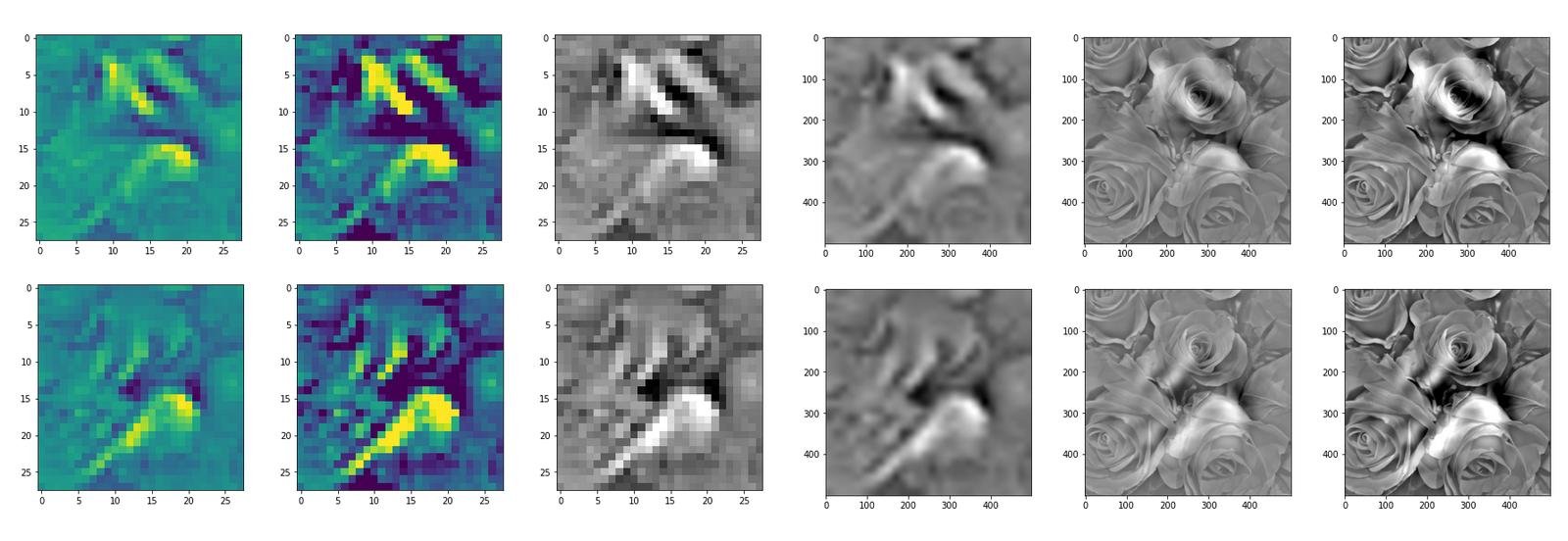“Donut …”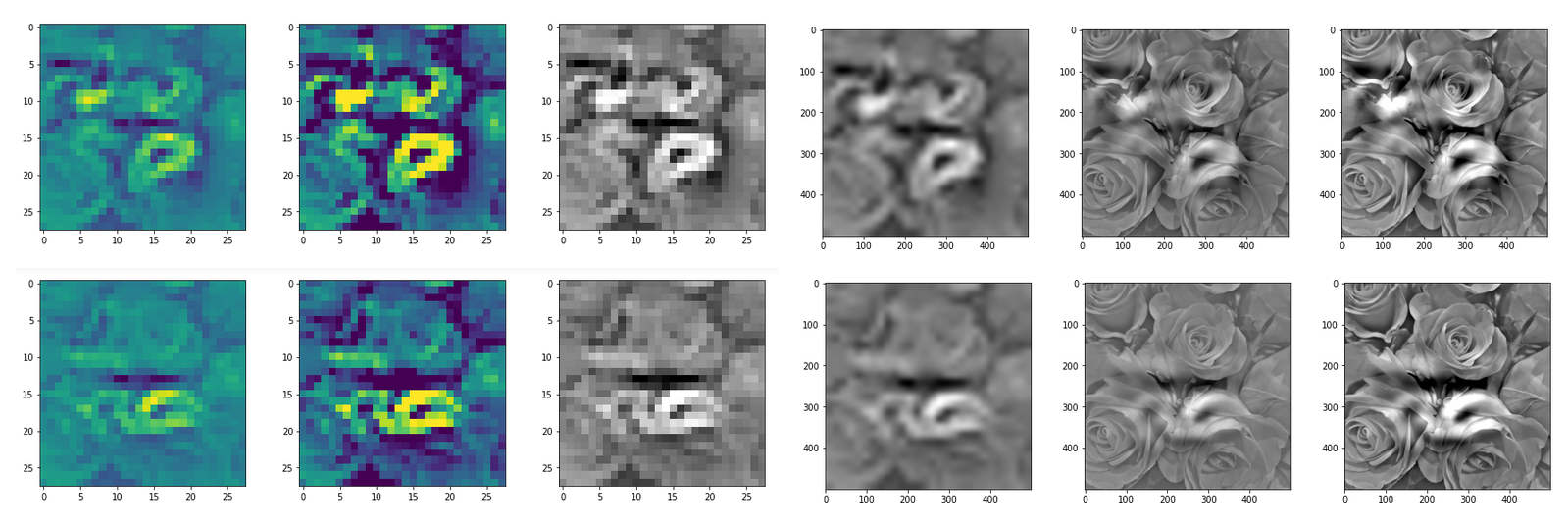“Curls to form a 3 …”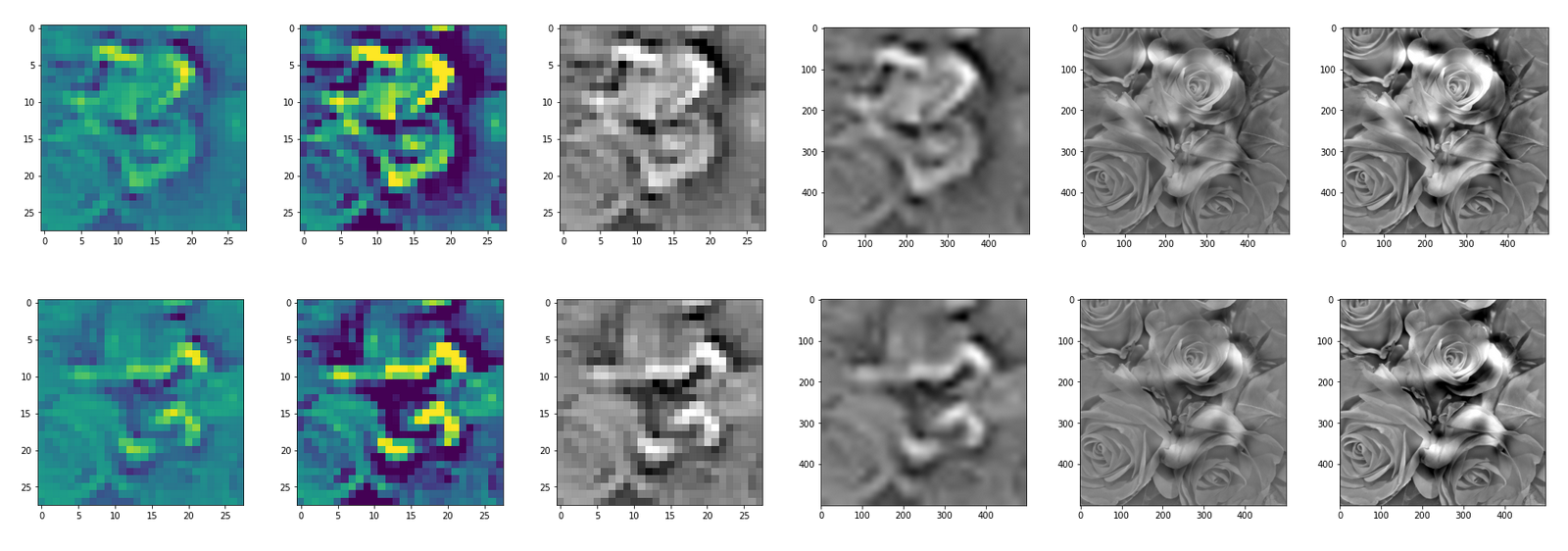“Two of them …”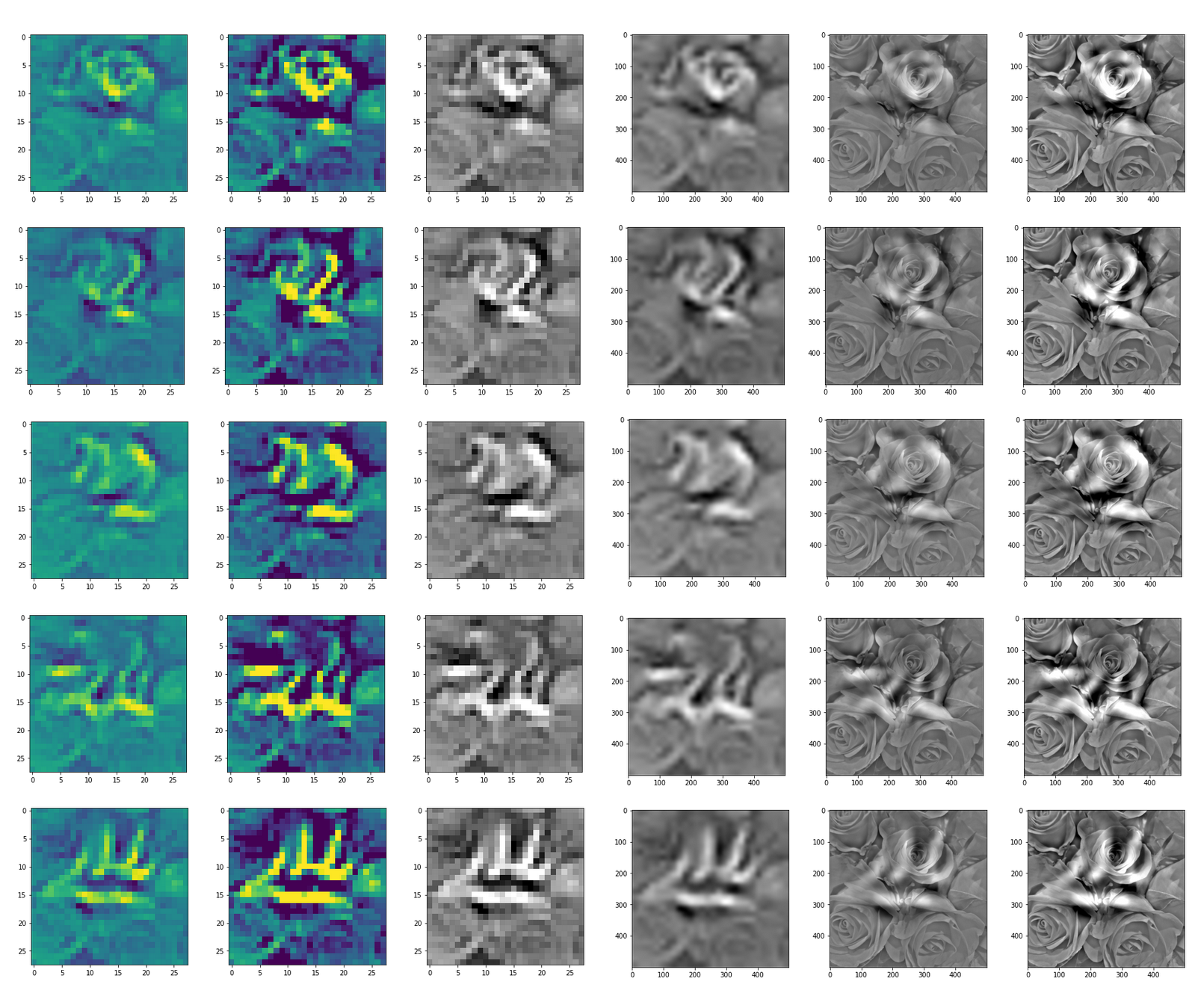“The creepy roots of it all …”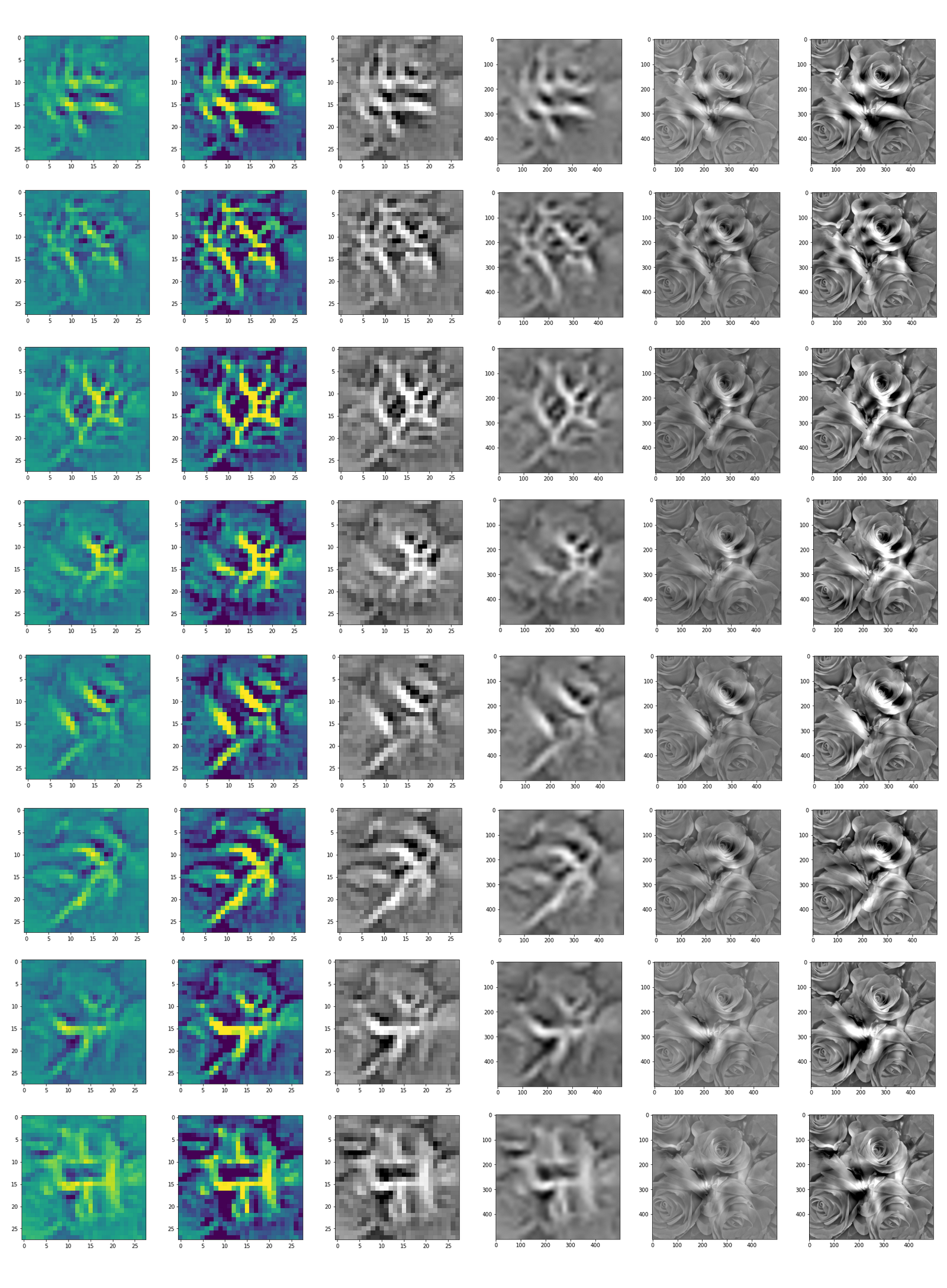“Look at me …”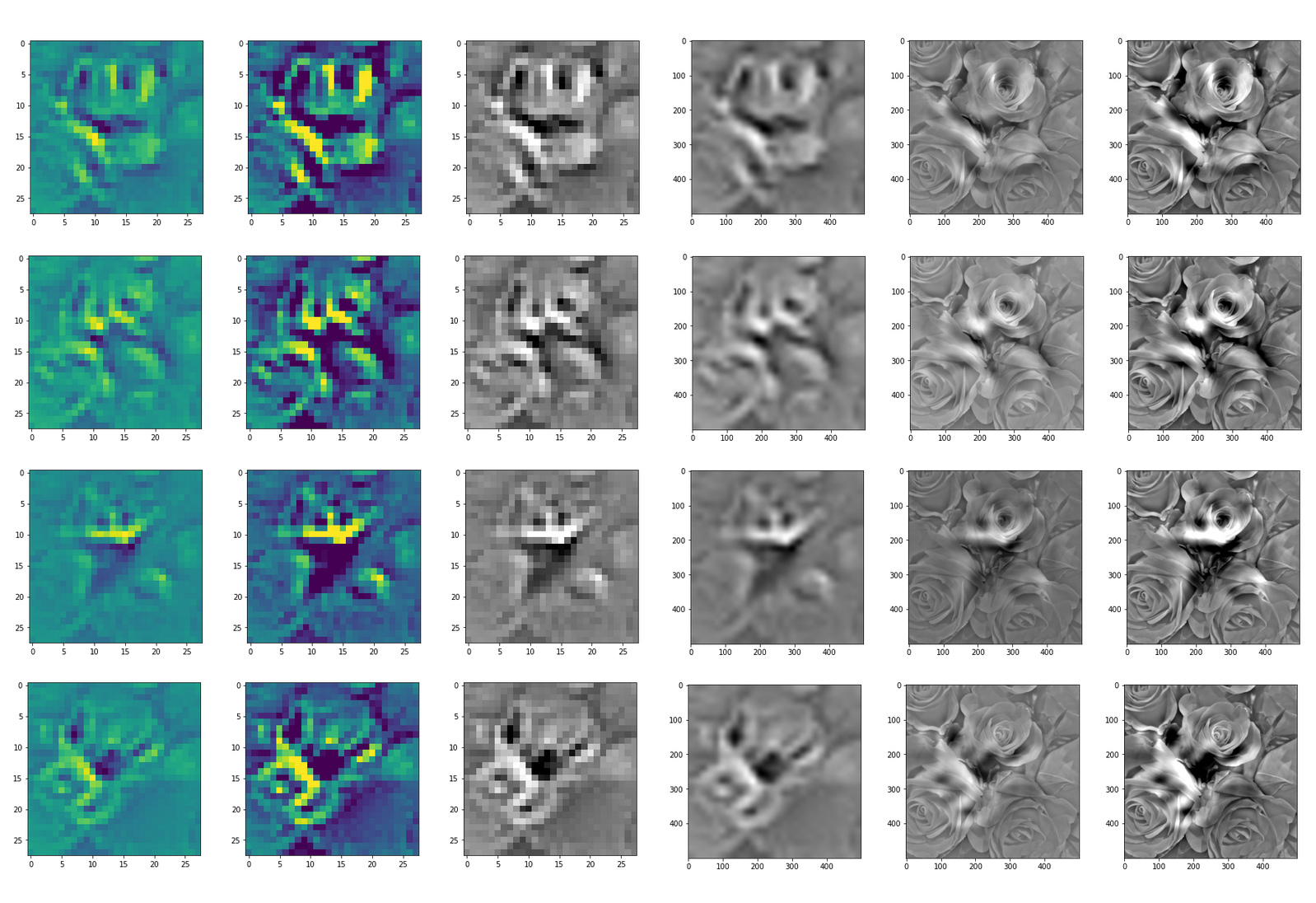“A hidden opening …”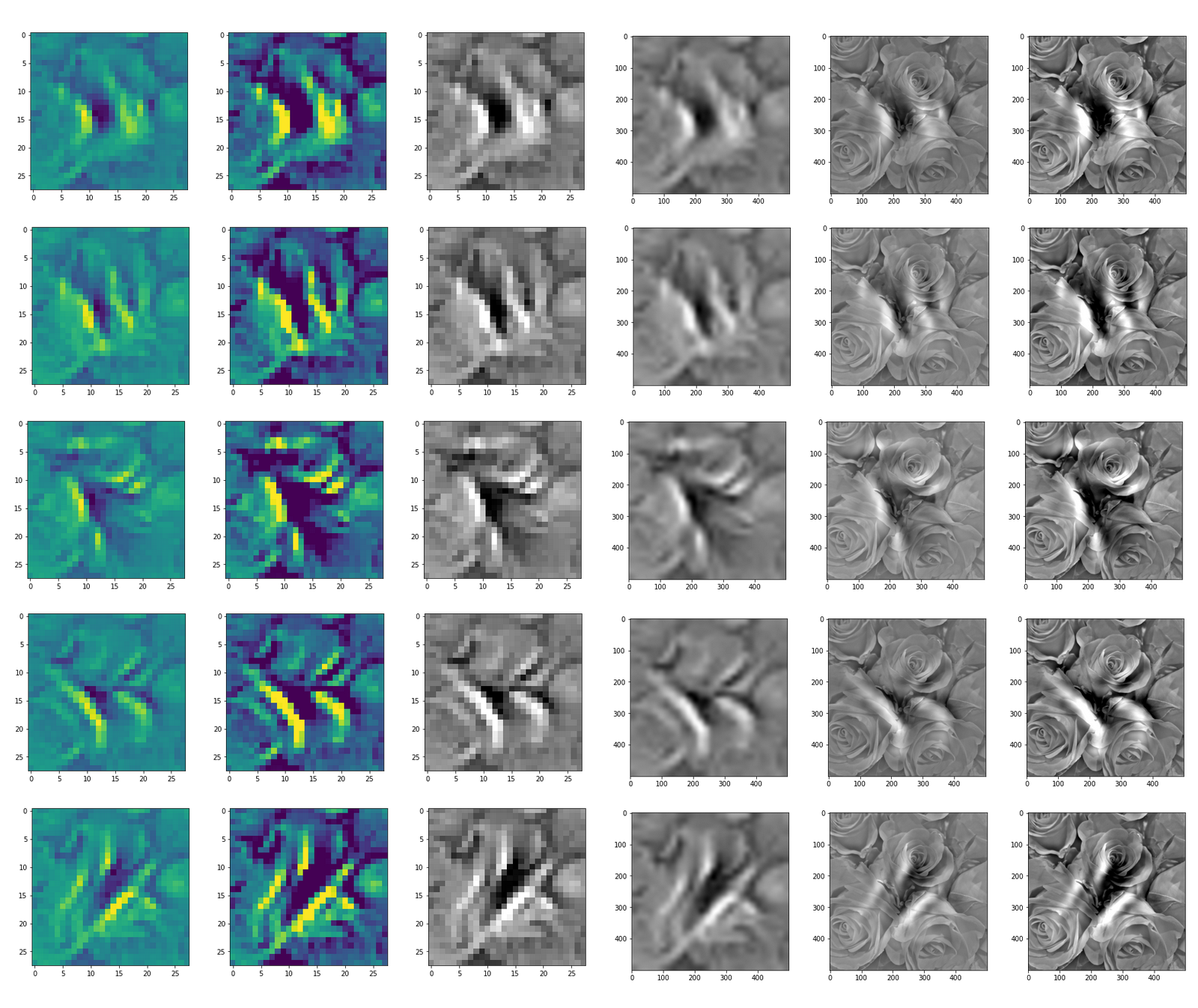“Soft is something different …”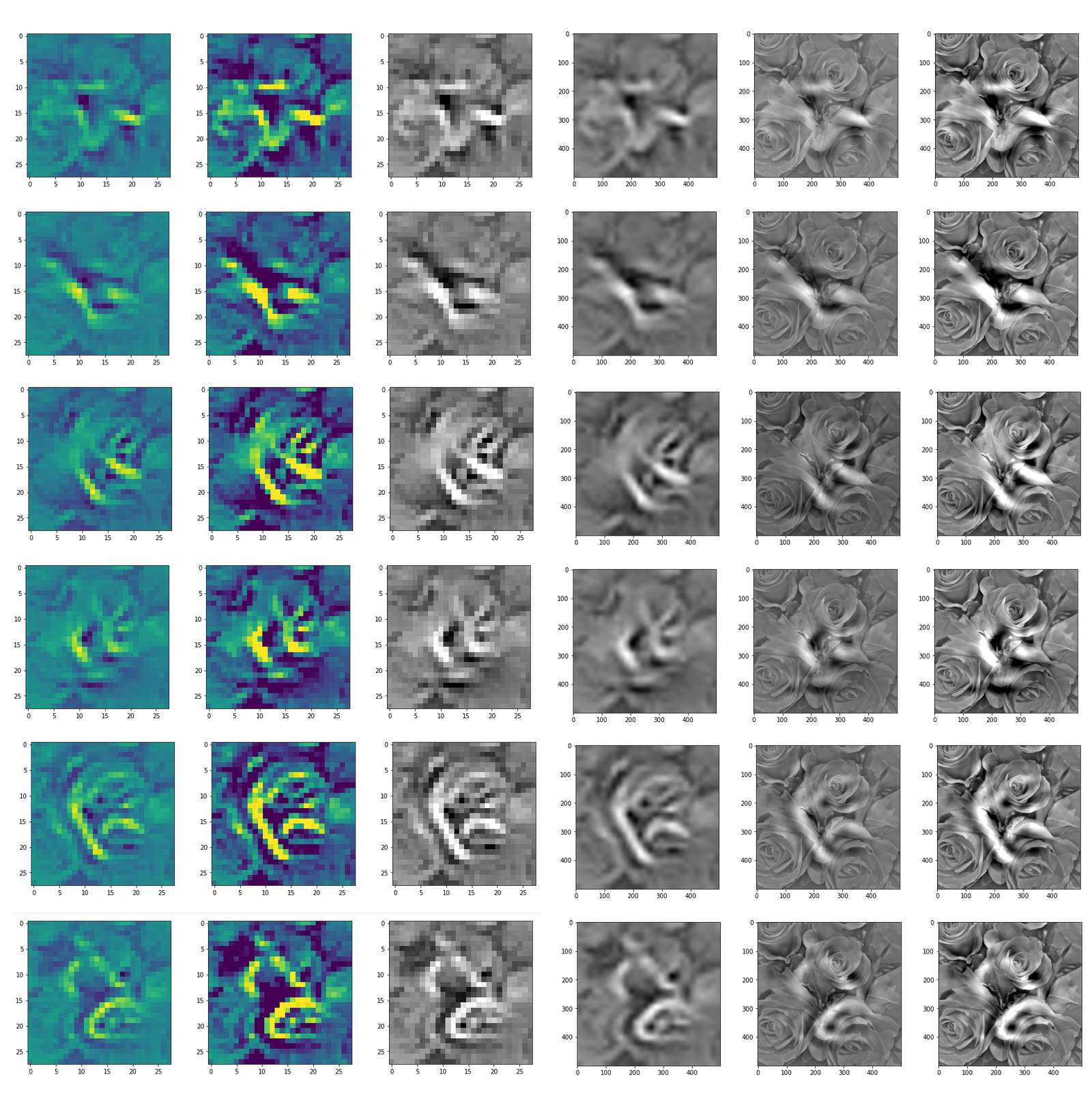“Central separation …”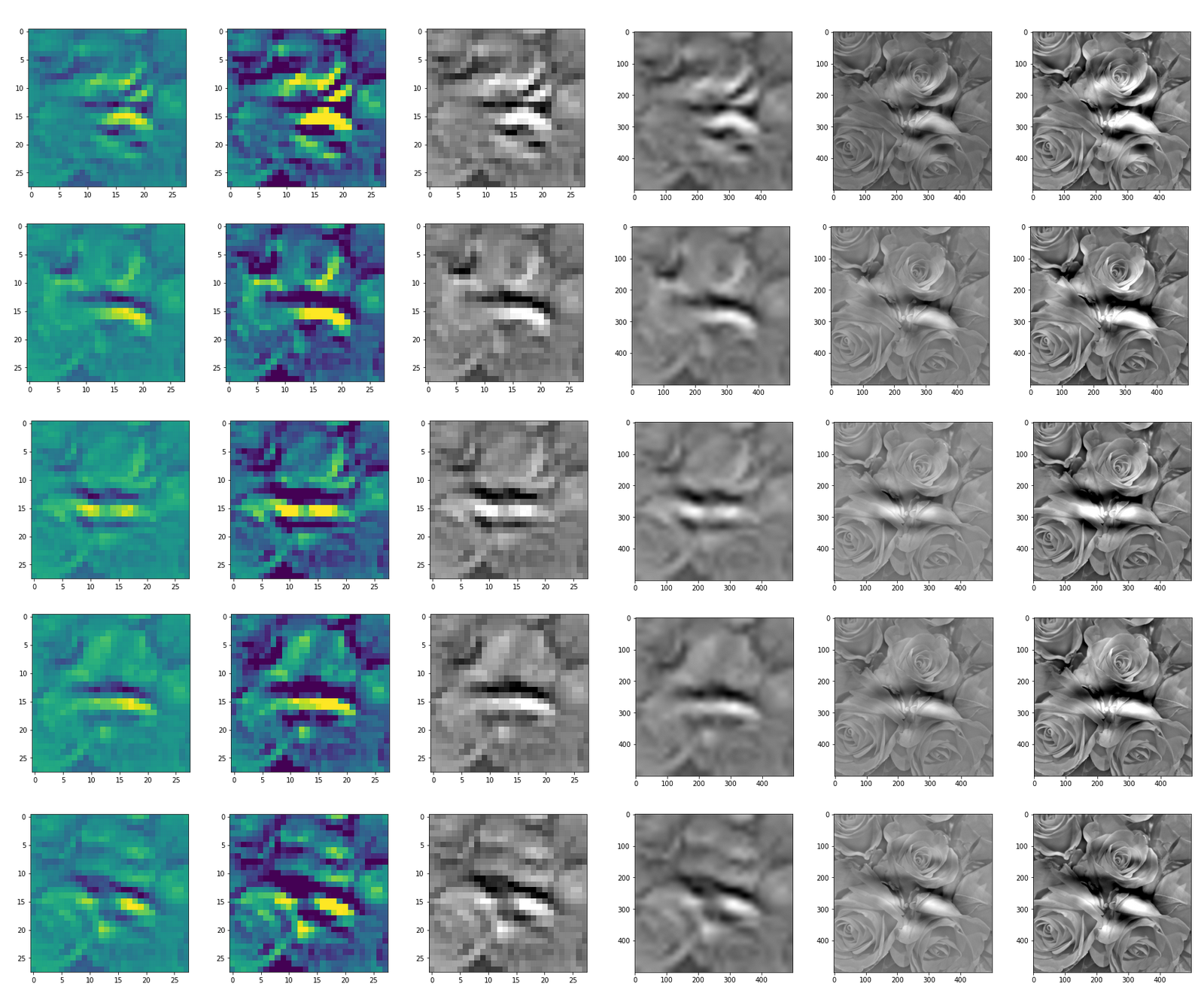# Conclusion: A CNN detects patterns or parts of patterns it was trained for in any kind of offered input …

You can compare the results to some input patterns (OIPs) which strongly trigger individual maps on the 3rd convolutional layer; you will detect similarities. E.g. four OIP- or feature patterns to which map 56 reacts strongly, look like:

This explains the basic shape of the “apparition” in the first “dream”: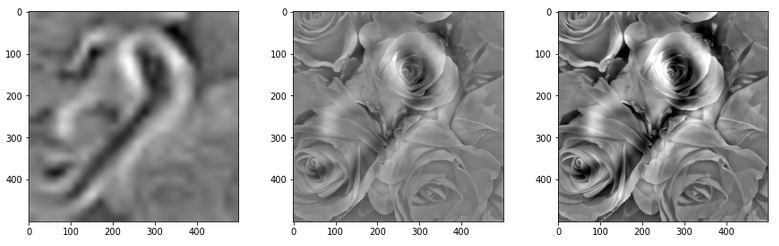This proves that the filters of a trained CNN actually detect patterns, which proved to be useful for a certain training purpose, in any kind of input which shows some traces of such patterns. A CNN simply does not “know” better: If you only have a hammer to interact with the world, everything becomes a nail to you in the end – this is the level of stupidity on which a CNN algorithm works. And it actually is a fundamental ingredient of DeepDream image manipulation – a transportation of learned patterns or prejudices to an environment outside the original training context.

In the next article
Deep Dreams of a CNN trained on MNIST data – II – some code for pattern carving
I provide the code for creating the above images.

# A simple CNN for the MNIST dataset – VI – classification by activation patterns and the role of the CNN’s MLP part

I continue with my series on a simple CNN used upon the MNIST dataset.

In the last article I discussed the following points:

• The series of convolutional transformations, which a CNN applies to its input, eventually leads to abstract representations in low dimensional parameter spaces, called maps. In the case of our CNN we got 128 (3×3)-maps at the last convolutional layer. 3×3 indeed means a very low resolution.
• We saw that the transformations would NOT produce results on the eventual maps which could be interpreted in the sense of figurative elements of depicted numbers, such as straight lines, circles or bows. Instead, due to pooling layers, lines and curved line elements obviously experience a fast dissolution during propagation through the various Conv layers. Whilst the first Conv layer still gives fair representations of e.g. a “4”, line-like structures get already unclear at the second Conv layer and more or less disappear at the maps of the last convolutional layer.
• This does not mean that a map on a deep convolutional layer does not react to some specific pattern within the pixel data of an input image. We called such patterns OIPs in last article and we were careful to describe them as geometrical correlations of pixels – and not conceptual entities. The sequence of convolutions which makes up a map on a deep convolutional layer corresponds to a specific combination of filters applied to the image data. This led us to the the theoretical idea that a map may indeed select a specific OIP in an input image and indicate the existence of such a OIP pattern by some activation pattern of the “neurons” within the map. However, we have no clue at the moment what such OIPs may look like and whether they correspond to conceptual entities which other authors usually call “features”.
• We saw that the common elements of the maps of multiple images of a handwritten “4” correspond to point-like activations within specific low dimensional maps on the output side of the last convolutional layer.
• The activations seem to form abstract patterns across the maps of the last convolutional layer. These patterns, which we called FCPs, seem to support classification decisions, which the MLP-part of the CNN has to make.

So, at our present level of the analysis of a CNN, we cannot talk in a well founded way about “features” in the sense of conceptual entities. We got, however, the impression that eventual abstractions of some patterns which are present in MNIST images of different digits lead to FCP patterns across maps which
allow for a classification of the images (with respect to the represented digits). We identified at least some common elements across the eventual maps of 3 different images of handwritten “4”s.

But it is really this simple? Can we by just looking for visible patterns in the activation output of the last convolutional layer already discriminate between different digits?

In this article I want to show that this is NOT the case. To demonstrate this we shall look at the image of a “4” which could also be almost classified to represent a “9”. We shall see

• that the detection of clear unique patterns becomes really difficult when we look at the representations of “4”s which almost resemble a “9” – at least from a human point of view;
• that directly visible patterns at the last convolutional layer may not contain sufficiently clear information for a classification;
• that the MLP part of our CNN nevertheless detects patterns after a linear transformation – i.e. after a linear combination of the outputs of the last Conv layer – which are not directly evident for human eyes. These “hidden” patterns do, however, allow for a rather solid classification.

# What have “4”s in common after three convolutional transformations?

As in the last article I took three clear “4” images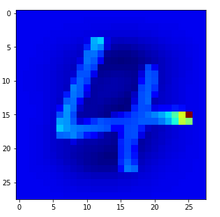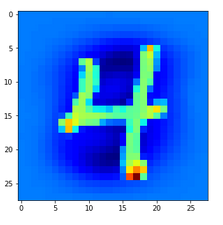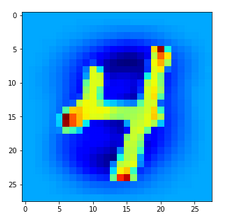and compared the activation output after three convolutional transformations – i.e. at the output side of the last Conv layer which we named “Conv2D_3”: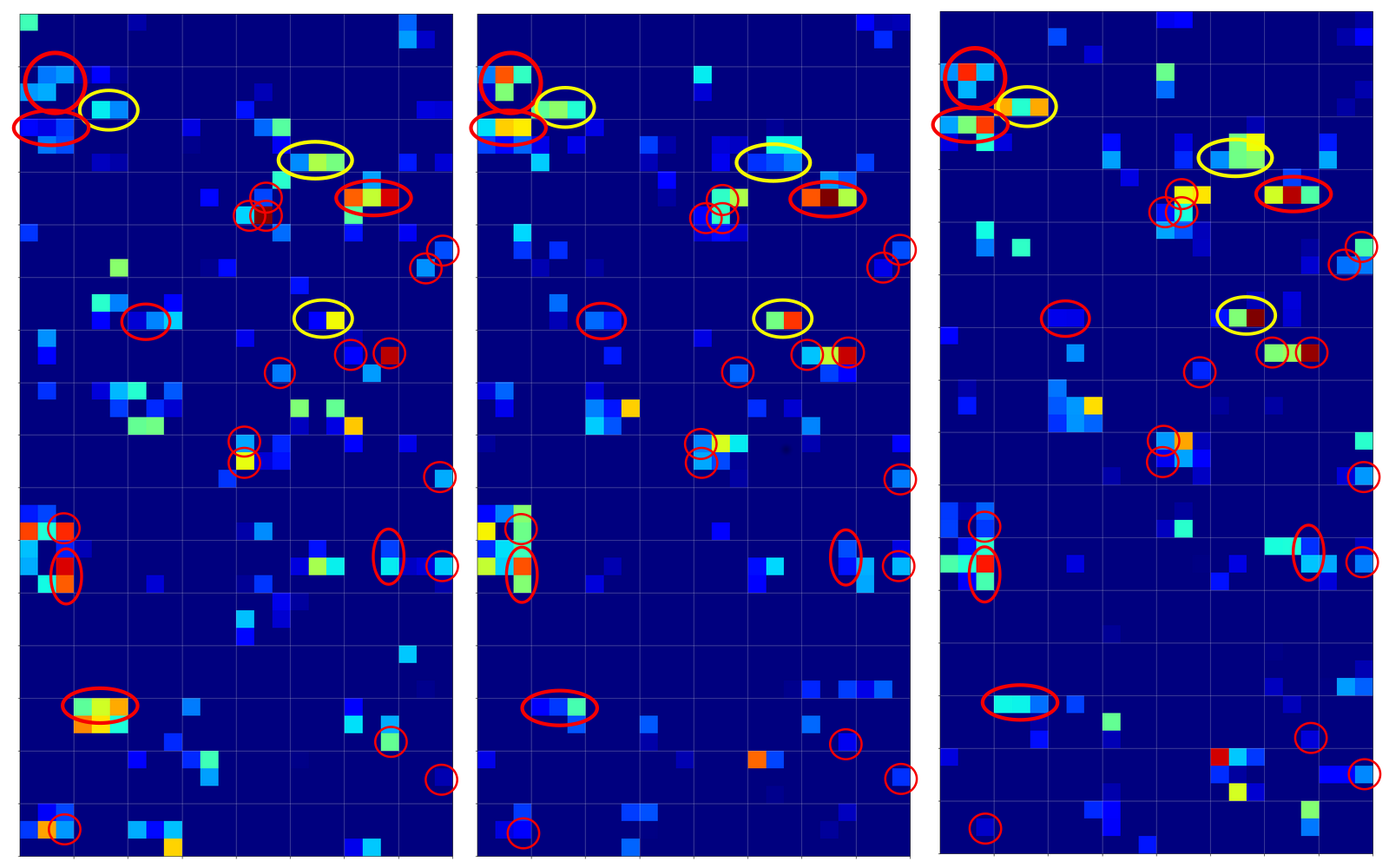The red circles indicate common points in the resulting 128 maps which we do not find in representations of three clear “9”s (see below). The yellow circles indicate common patterns which, however, appear in some representations of a “9”.

# What have “9”s in common after three convolutional transformations?

Now let us look at the same for three clear “9”s: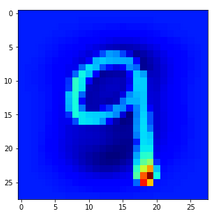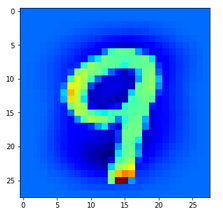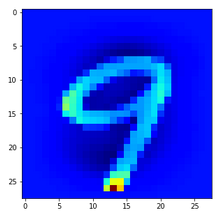A comparison gives the following common features of “9”s on the third Conv2D layer: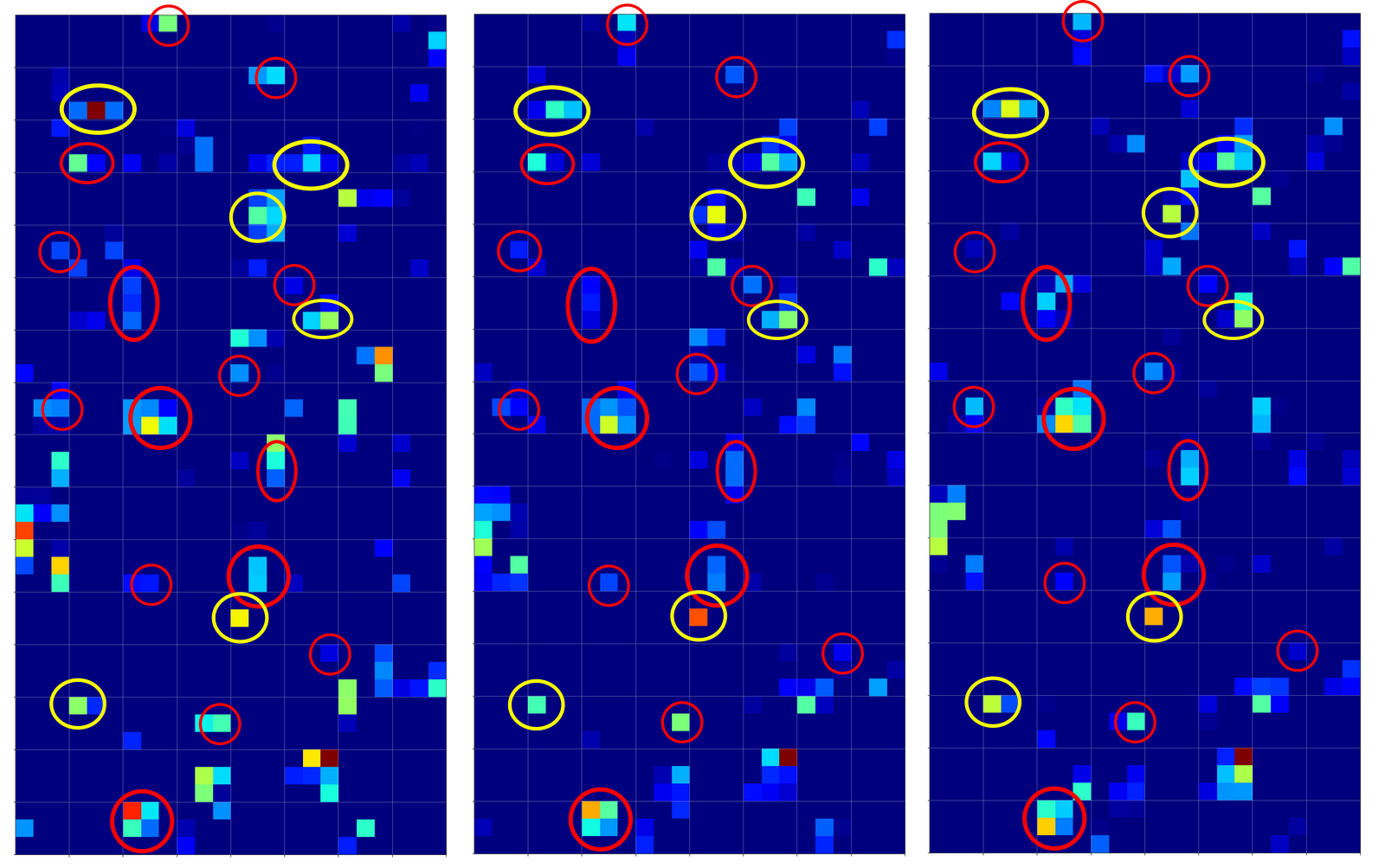We again get the impression that enough unique features seem to exist on the maps for “4”s and “9”s, respectively, to distinguish between images of these numbers. But is it really so simple?

# Intermezzo: Some useful steps to reproduce results

You certainly do not want to perform a training all the time when you want to analyze predictions at certain layers for some selected MNIST images. And you may also need the same “X_train”, “X_test” sets to identify one and the same image by a defined number. Remember: In the Python code which I presented in a previous article for the setup for the data samples no unique number would be given due to initial shuffling.

Thus, you may need to perform a training run and then save the model as well as your X_train, y_train and X_test, y_test datasets. Note that we have transformed the data already in a reasonable tensor form which Keras expects. We also had already used one-hot-labels. The transformed sets were called “train_imgs”, “test_imgs”, “train_labels”, “test_labels”, “y_train”, “y_test”

The following code saves the model (here “cnn”) at the end of a training and loads it again:

```# save a full model
cnn.save('cnn.h5')

```

On a Linux system the default path is typically that one where you keep your Jupyter notebooks.

The following statements save the sets of tensor-like image data in Numpy compatible data (binary) structures:

```# Save the data

from numpy import save
save('train_imgs.npy', train_imgs)
save('test_imgs.npy', test_imgs)
save('train_labels.npy', train_labels)
save('test_labels.npy', test_labels)
save('y_train.npy', y_train)
save('y_test.npy', y_test)
```

```# Load train, test image data (in tensor form)

```

Be careful to save only once – and not to set up and save your training and test data again in a pure analysis session! I recommend to use different notebooks for training and later analysis. If you put all your code in just one notebook you may accidentally run Jupyter cells again, which you do not want to run during analysis sessions.

# What happens for unclear representations/images of a “4”?

When we trained a pure MLP on the MNIST dataset we had a look at the confusion matrix:
A simple Python program for an ANN to cover the MNIST dataset – XI – confusion matrix.
We saw that the MLP e.g. confused “5”s with “9s”, “9”s with “4”s, “2”s with “7”s, “8”s with “5”s – and vice versa. We got the highest confusion numbers for the misjudgement of badly written “4”s and “9”s.

Let us look at a regular 4 and two “4”s which with some good will could also be interpreted as representations of a “9”; the first one has a closed upper area – and there are indeed some representations of “9”s in the MNIST dataset which look similar. The second “4” in my view is even closer to a “9”: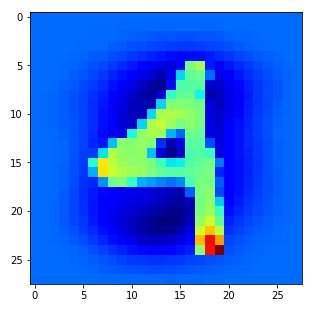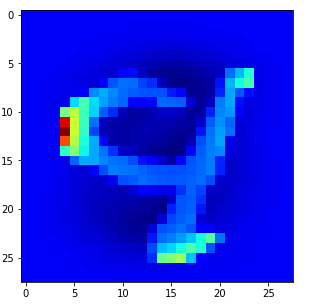Now, if we wanted to look out for the previously discussed “unique” features of “4”s and “9s” we would get a bit lost: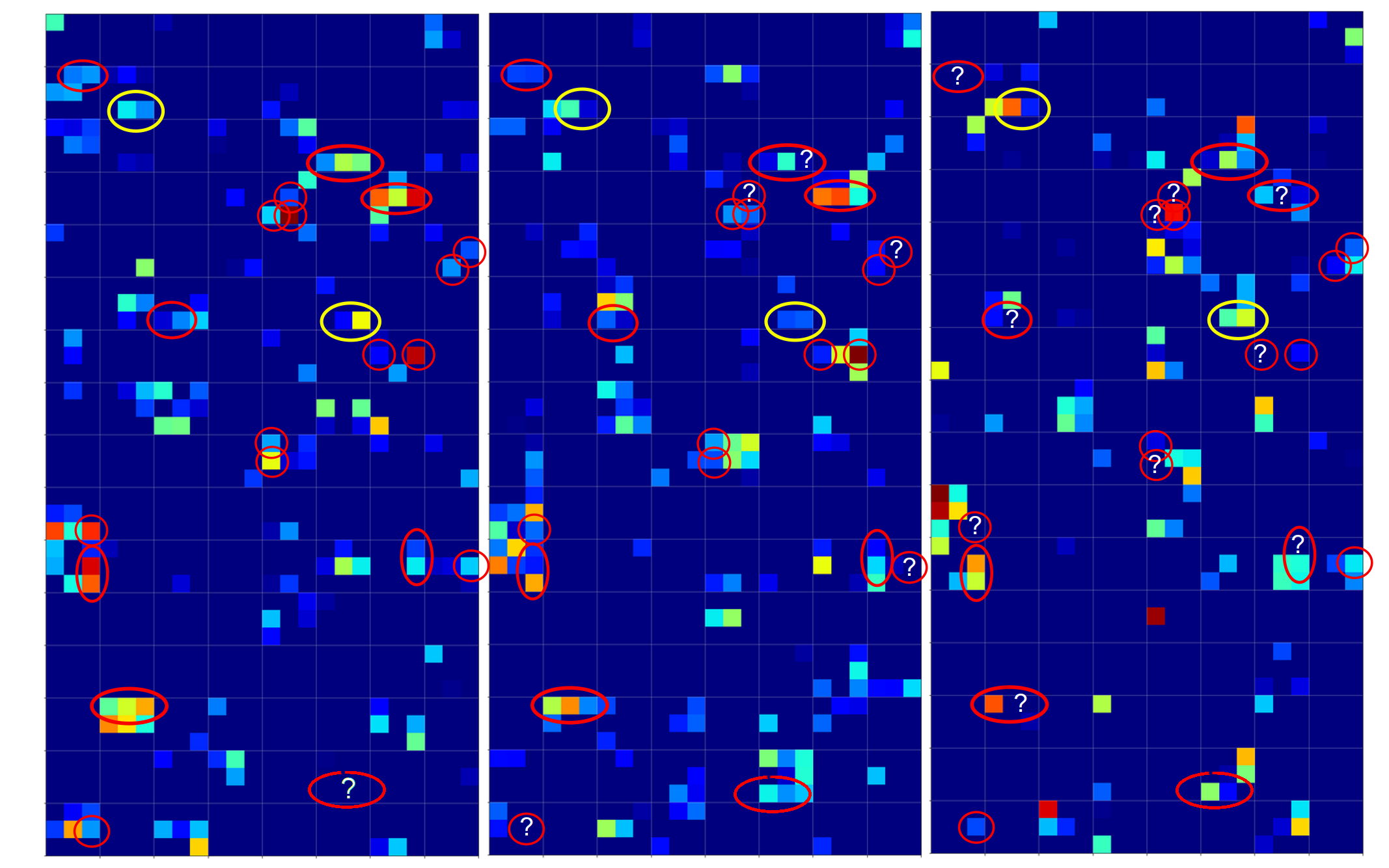The first image is for a clear “4”. The last two are the abstractions for our two newly chosen unclear “4”s in the order given above.

You see: Many of our seemingly “unique features” for a “4” on the third Conv-level are no longer or not fully present for our second “4”; so we would be rather insecure if we had to judge the abstraction as a viable pattern for a “4”. We would expect that this “human” uncertainty also shows up in a probability distribution at the output layer of our CNN.

But, our CNN (including its MLP-part) has no doubt about the classification of the last sample as a “4”. We just look at the prediction output of our model:

```# Predict for a single image
# ****************************
num_img = 1302
ay_sgl_img = test_imgs[num_img:num_img+1]
print(ay_sgl_img.shape)
# load last cell for the next statement to work
#prob = cnn_pred.predict_proba(ay_sgl_img, batch_size=1)
#print(prob)
prob1 = cnn_pred.predict(ay_sgl_img, batch_size=1)
print(prob1)

[[3.61540742e-07 1.04205284e-07 1.69877489e-06 1.15337198e-08
9.35641170e-01 3.53500056e-08 1.29525617e-07 2.28584581e-03
2.59062881e-06 6.20680153e-02]]

```

93.5% probability for a “4”! A very clear discrimination! How can that be, given the – at first sight – seemingly unclear pattern situation at the third activation layer for our strange 4?

# The MLP-part of the CNN “sees” things we humans do not see directly

We shall not forget that the MLP-part of the CNN plays an important role in our game. It reduces the information of the last 128 maps (3x3x128 = 1152) values down to 100 node values with the help of 115200 distinguished weights for related connections. This means there is a lot of fine-tuned information extraction and information compactification going on at the border of the CNN’s MLP part – a transformation step which is too complex to grasp directly.

It is the transformation of all the 128x3x3-map-data into all 100 nodes via a linear combination which makes things difficult to understand. 115200 optimized weights leave enough degrees of freedom to detect combined patterns in the activation data which are more complex and less obvious than the point-like structures we encircled in the images of the maps

So, it is interesting to visualize and see how the MLP part of our CNN reacts to the activations of the last convolutional layers. Maybe we find some more intriguing patterns there, which discriminate “4”s from “9”s and explain the rather clear probability evaluation.

# Visualization of the output of the dense layers of the CNN’s MLP-part

We need to modify some parts of our code for creating images of the activation outputs of convolutional layers to be able to produce equally reasonable images for the output of the dense MLP layers, too. These modifications are simple. We distinguish between the types of layers by their names: When the name contains “dense” we execute a slightly different code. The changes affect just the function “img_grid_of_layer_activation()” previously discussed as the contents of a Jupyter “cell 9“:

```
# Function to plot the activations of a layer
# --------------------------
-----------------
# Adaption of a method originally designed by F.Chollet

def img_grid_of_layer_activation(d_img_sets, model_fname='cnn.h5', layer_name='', img_set="test_imgs", num_img=8,
scale_img_vals=False):
'''
Input parameter:
-----------------
d_img_sets: dictionary with available img_sets, which contain img tensors (presently: train_imgs, test_imgs)
model_fname: Name of the file containing the models data
layer_name: name of the layer for which we plot the activation; the name must be known to the Keras model (string)
image_set: The set of images we pick a specific image from (string)
num_img: The sample number of the image in the chosen set (integer)
scale_img_vals: False: Do NOT scale (standardize) and clip (!) the pixel values. True: Standardize the values. (Boolean)

Hints:
-----------------
We assume quadratic images - in case of dense layers we assume a size of 1
'''

# get the output of a certain named layer - this includes all maps
# https://keras.io/getting_started/faq/#how-can-i-obtain-the-output-of-an-intermediate-layer-feature-extraction
cnnx_layer_output = cnnx.get_layer(layer_name).output

# build a new model for input "cnnx.input" and output "output_of_layer"
# ~~~~~~~~~~~~~~~~~
# Keras knows the required connections and intermediat layers from its tensorflow graphs - otherwise we get an error
# The new model can make predictions for a suitable input in the required tensor form
mod_lay = models.Model(inputs=cnnx.input, outputs=cnnx_layer_output)

# Pick the input image from a set of respective tensors
if img_set not in d_img_sets:
print("img set " + img_set + " is not known!")
sys.exit()
# slicing to get te right tensor
ay_img = d_img_sets[img_set][num_img:(num_img+1)]

# Use the tensor data as input for a prediction of model "mod_lay"
lay_activation = mod_lay.predict(ay_img)
print("shape of layer " + layer_name + " : ", lay_activation.shape )

# number of maps of the selected layer
n_maps   = lay_activation.shape[-1]
print("n_maps = ", n_maps)

# size of an image - we assume quadratic images
# in the case  of "dense" layers we assume that the img size is just 1 (1 node)
if "dense" in layer_name:
img_size = 1
else:
img_size = lay_activation.shape
print("img_size = ", img_size)

# Only for testing: plot an image for a selected
# map_nr = 1
#plt.matshow(lay_activation[0,:,:,map_nr], cmap='viridis')

# We work with a grid of images for all maps
# ~~~~~~~~~~~~~~~----------------------------
# the grid is build top-down (!) with num_cols and num_rows
# dimensions for the grid
num_imgs_per_row = 8
num_cols = num_imgs_per_row
num_rows = n_maps // num_imgs_per_row
#print("img_size = ", img_size, " num_cols = ", num_cols, " num_rows = ", num_rows)

# grid
dim_hor = num_imgs_per_row * img_size
dim_ver = num_rows * img_size
img_grid = np.zeros( (dim_ver, dim_hor) )   # horizontal, vertical matrix
print("shape of img grid = ", img_grid.shape)

# double loop to fill the grid
n = 0
for row in range(num_rows):
for col in range(num_cols):
n += 1
#print("n = ", n, "row = ", row, " col = ", col)
# in case of a dense layer the shape of the tensor like output
# is different in comparison to Conv2D layers
if "dense" in layer_name:
present_img = lay_activation[ :, row*num_imgs_per_row + col]
else:

present_img = lay_activation[0, :, :, row*num_imgs_per_row + col]

# standardization and clipping of the img data
if scale_img_vals:
present_img -= present_img.mean()
if present_img.std() != 0.0: # standard deviation
present_img /= present_img.std()
#present_img /= (present_img.std() +1.e-8)
present_img *= 64
present_img += 128
present_img = np.clip(present_img, 0, 255).astype('uint8') # limit values to 255

# place the img-data at the right space and position in the grid
# ~~~~~~~~~~~~~~~~~~~~~~~~~~~~~~~~~~~~~~~~~~~~~~~~~~~~~~~~~~~~~~
# the following is only used if we had reversed vertical direction by accident
#img_grid[row*img_size:(row+1)*(img_size), col*img_size:(col+1)*(img_size)] = np.flip(present_img, 0)
img_grid[row*img_size:(row+1)*(img_size), col*img_size:(col+1)*(img_size)] = present_img

return img_grid, img_size, dim_hor, dim_ver
```

You certainly detect the two small changes in comparison to the code for Jupyter cell 9 of the article
A simple CNN for the MNIST dataset – IV – Visualizing the output of convolutional layers and maps.

However, there remains one open question: We were too lazy in the coding discussed in previous articles to create our own names names for the dense layers. This is, however, no major problem: Keras creates its own names – if we do not define our own layer names when constructing a CNN model. Where do we get these default names from? Well, from the model’s summary:

```cnn_pred.summary()

Model: "sequential_7"
_________________________________________________________________
Layer (type)                 Output Shape              Param #
=================================================================
Conv2D_1 (Conv2D)            (None, 26, 26, 32)        320
_________________________________________________________________
Max_Pool_1 (MaxPooling2D)    (None, 13, 13, 32)        0
_________________________________________________________________
Conv2D_2 (Conv2D)            (None, 11, 11, 64)        18496
_________________________________________________________________
Max_Pool_2 (MaxPooling2D)    (None, 5, 5, 64)          0
_________________________________________________________________
Conv2D_3 (Conv2D)            (None, 3, 3, 128)         73856
_________________________________________________________________
flatten_7 (Flatten)          (None, 1152)              0
_________________________________________________________________
dense_14 (Dense)             (None, 100)               115300
_________________________________________________________________
dense_15 (Dense)             (None, 10)                1010
=================================================================
Total params: 208,982
Trainable params: 208,982
Non-trainable params: 0
_________________________________________________________________

```

Our first MLP layer with 100 nodes obviously got the name “dense_14”.

With our modification and the given name we can now call Jupyter “cell 10” as before:

```
# Plot the img grid of a layers activation
# ~~~~~~~~~~~~~~~~~~~~~~~~~~~~~~~~~~~~~~~~~

# global dict for the image sets
d_img_sets= {'train_imgs':train_imgs, 'test_imgs':test_imgs}

# layer - pick one of the names which you defined for your model
layer_name = "dense_14"

# choose a image_set and an img number
img_
set = "test_imgs"

# clear 4
num_img = 1816

#unclear 4
#num_img = 1270
#num_img = 1302

#clear 9
#num_img = 1249
#num_img = 1410
#num_img = 1858

# Two figures
# -----------
fig1 = plt.figure(1, figsize=(5,5))  # figure for the input img
fig2 = plt.figure(2)  # figure for the activation outputs of th emaps

fig1 = plt.figure( figsize=(5,5) )
ay_img = test_imgs[num_img:num_img+1]
#plt.imshow(ay_img[0,:,:,0], cmap=plt.cm.binary)
plt.imshow(ay_img[0,:,:,0], cmap=plt.cm.jet)

# getting the img grid
img_grid, img_size, dim_hor, dim_ver = img_grid_of_layer_activation(
d_img_sets, model_fname='cnn.h5', layer_name=layer_name,
img_set=img_set, num_img=num_img,
scale_img_vals=False)
# Define reasonable figure dimensions by scaling the grid-size
scale = 1.6 / (img_size)
fig2 = plt.figure( figsize=(scale * dim_hor, scale * dim_ver) )
#axes
ax = fig2.gca()
ax.set_xlim(-0.5,dim_hor-1.0)
ax.set_ylim(dim_ver-1.0, -0.5)  # the grid is oriented top-down
#ax.set_ylim(-0,dim_ver-1.0) # normally wrong

# setting labels - tick positions and grid lines
ax.set_xticks(np.arange(img_size-0.5, dim_hor, img_size))
ax.set_yticks(np.arange(img_size-0.5, dim_ver, img_size))
ax.set_xticklabels([]) # no labels should be printed
ax.set_yticklabels([])

# preparing the grid
plt.grid(b=True, linestyle='-', linewidth='.5', color='#ddd', alpha=0.7)

# color-map
#cmap = 'viridis'
#cmap = 'inferno'
cmap = 'jet'
#cmap = 'magma'

plt.imshow(img_grid, aspect='auto', cmap=cmap)
```

In the output picture each node will be represented by a colored rectangle.

# Visualization of the output for clear “4”s at the first dense MLP-layer

The following picture displays the activation values for three clear “4”s at the first dense MLP layer: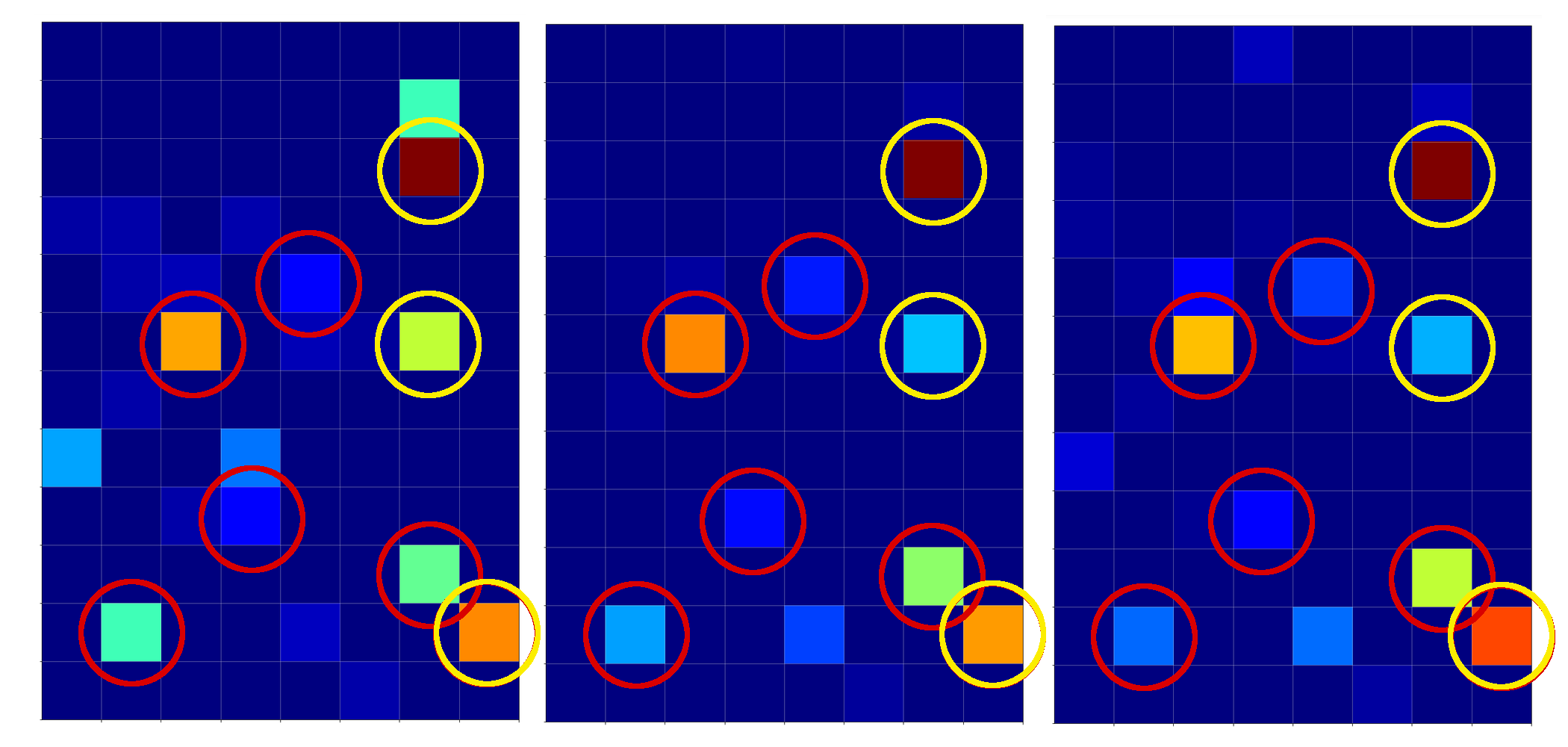I encircled again some of the nodes which carry some seemingly “unique” information for representations of the digit “4”.

For clear “9”s we instead get: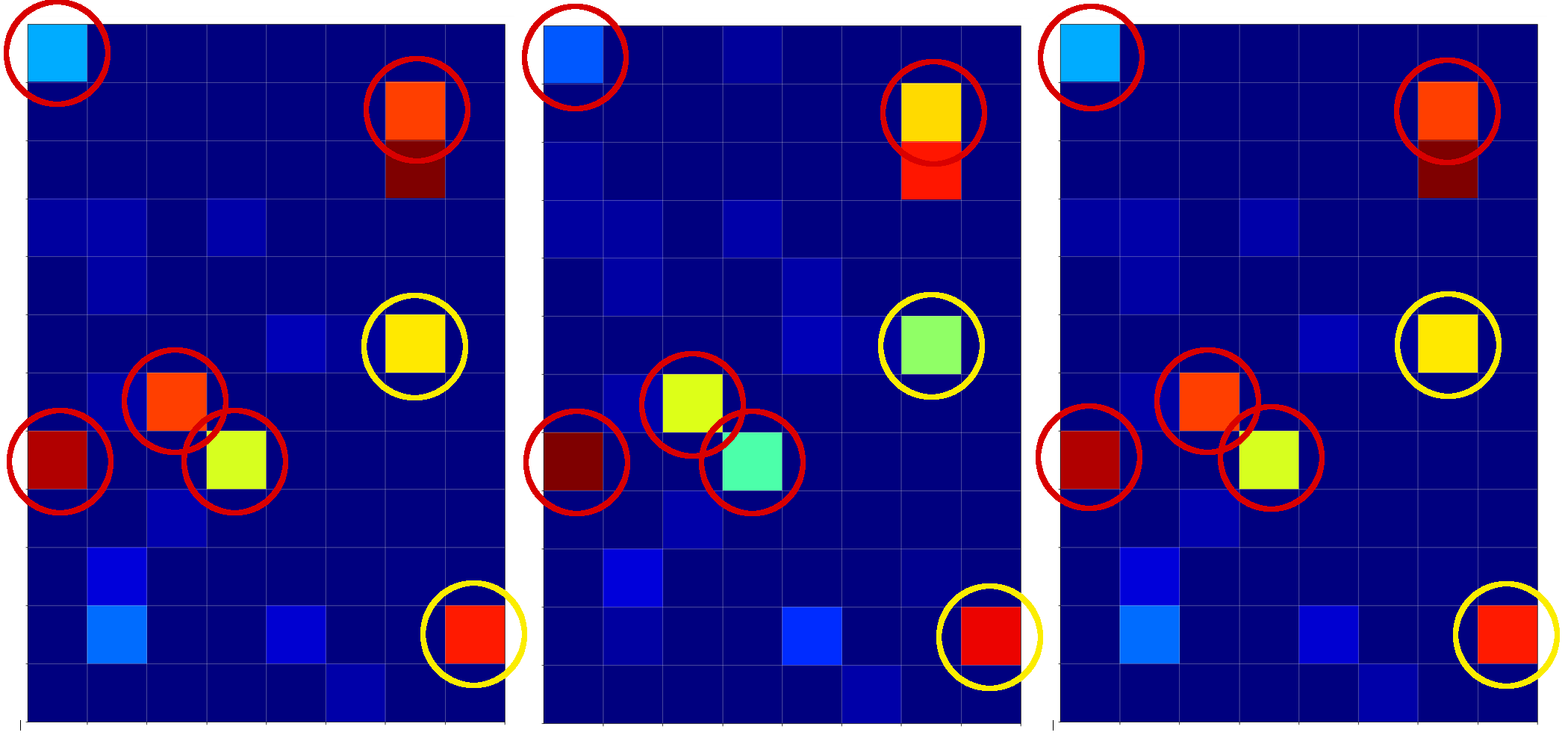Hey, there are some clear differences: Especially, the diagonal pattern (vertically a bit below the middle and horizontally a bit to the left) and the activation at the first node (upper left) seem to be typical for representations of a “9”.

# Our unclear “4” representations at the first MLP layer

Now, what do we get for our two unclear “4”s?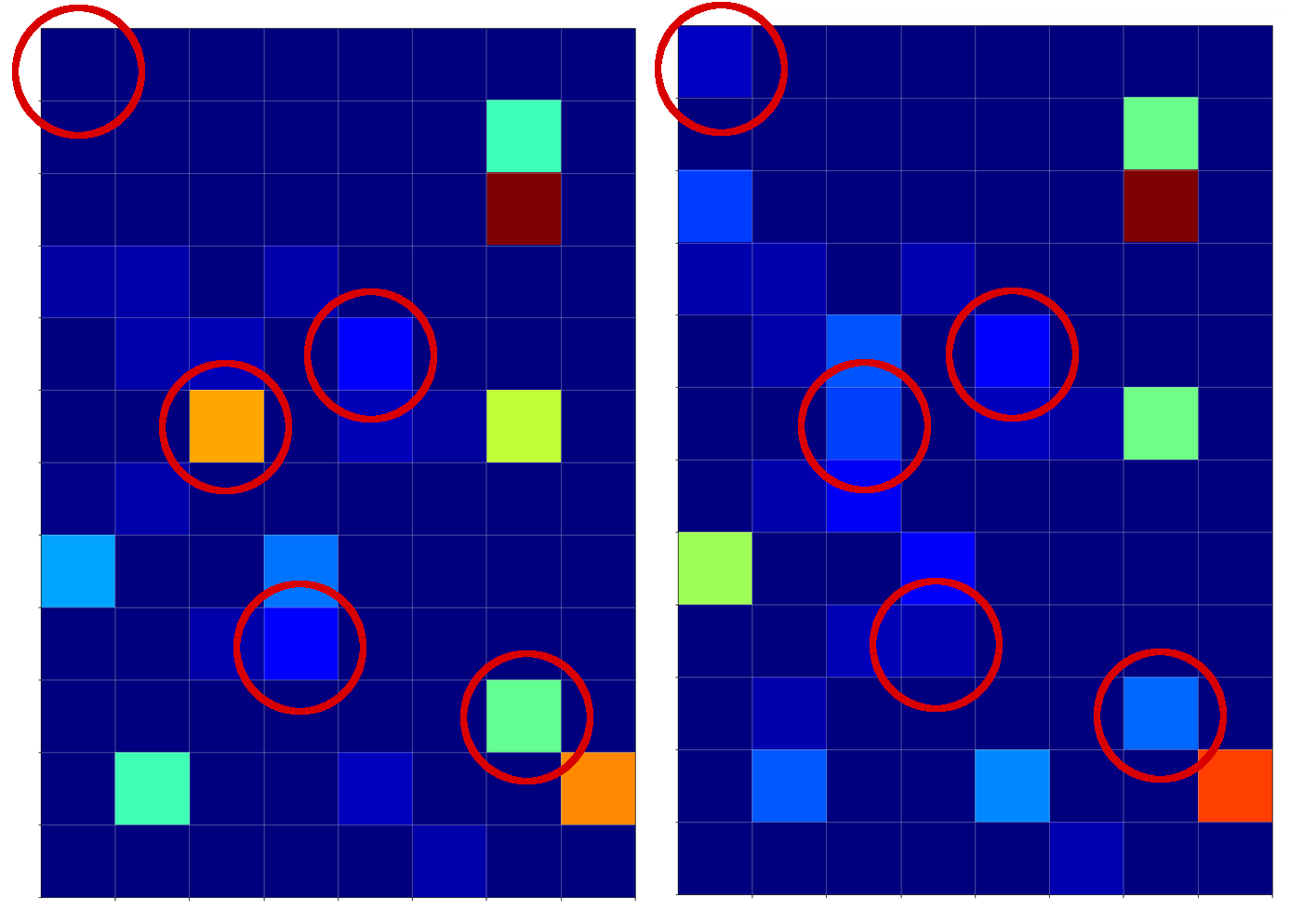I think that we would guess with confidence that our first image clearly corresponds to a “4”. With the second one we would be a bit more careful – but the lack of the mentioned diagonal structure with sufficiently high values (orange to yellow on the “jet”-colormap) would guide us to a “4”. Plus the presence of a relatively high value at a node present at the lower right which is nowhere in the “9” representations. Plus too small values at the upper left corner. Plus some other aspects – some nodes have a
value where all the clear “9”s do not have anything.

We should not forget that there are more than 1000 weights again to emphasize some combinations and suppress others on the way to the output layer of the CNN’s MLP part.

# Conclusion

Information which is still confusing at the last convolutional layer – at least from a human visual perspective – can be “clarified” by a combination of the information across all (128) maps. This is done by the MLP transformations (linear matrix plus non-linear activation function) which produce the output of the 1st dense layer.

Thus and of course, the dense layers of the MLP-part of a CNN play an important role in the classification process: The MLP may detect patterns in the the combined information of all available maps at the last convolutional layer which the human eye may have difficulties with.

In the sense of a critical review of the results of our last article we can probably say: NOT the individual points, which we marked in the images of the maps at the last convolutional layer, did the classification trick; it was the MLP analysis of the interplay of the information across all maps which in the end lead the CNN to an obviously correct classification.

Common features in calculated maps for MNIST images are nice, but without an analysis of a MLP across all maps they are not sufficient to solve the classification problem. So: Do not underestimate the MLP part of a CNN!

In the next article

A simple CNN for the MNIST dataset – VII – outline of steps to visualize image patterns which trigger filter maps

I shall outline some required steps to visualize the patterns or structures within an input image which a specific CNN map reacts to. This will help us in the end to get a deeper understanding of the relation between FCPs and OIPs. I shall also present some first images of such OIP patterns or “features” which activate certain maps of our trained CNN.

# A simple CNN for the MNIST dataset – IV – Visualizing the activation output of convolutional layers and maps

In the first three articles of this series on a (very) simple CNN for the MNIST dataset

we invested some work into building layers and into the parameterization of a training run. Our rewards comprised a high accuracy value of around 99.35% and watching interactive plots during training.

But a CNN offers much more information which is worth and instructive to look at. In the first article I have talked a bit about feature detection happening via the “convolution” of filters with the original image data or the data produced at feature maps of previous layers. What if we could see what different filters do to the underlying data? Can we have a look at the output selected “feature maps” produce for a specific input image?

Yes, we can. And it is intriguing! The objective of this article is to plot images of the feature map output at a chosen convolutional or pooling layer of our CNN. This is accompanied by the hope to better understand the concept of abstract features extracted from an input image.

I follow an original idea published by F. Chollet (in his book “Deep Learning mit Python und Keras”, mitp Verlag) and adapt it to the code discussed in the previous articles.

# Referring to inputs and outputs of models and layers

So far we have dealt with a complete CNN with a multitude of layers that produce intermediate tensors and a “one-hot”-encoded output to indicate the prediction for a hand-written digit represented by a MNIST image. The CNN itself was handled by Keras in form of a sequential model of defined convolutional and pooling layers plus layers of a multi-layer perceptron [MLP]. By the definition of such a “model” Keras does all the work required for forward and backward propagation steps in the background. After training we can “predict” the outcome for any new digit image which we feed into the CNN: We just have to fetch the data form th eoutput layer (at the end of the MLP) after a forward propagation with the weights optimized during training.

But now, we need something else:

We need a model which gives us the output, i.e. a 2-dimensional tensor – of a specific map of an intermediate Conv-layer as a prediction for an input image!

I.e. we want the output of a sub-model of our CNN containing only a part of the layers. How can we define such an (additional) model based on the layers of our complete original CNN-model?

Well, with Keras we can build a general model based on any (partial) graph of connected layers which somebody has set up. The input of such a model must follow rules appropriate to the receiving layer and the output can be that of a defined subsequent layer or map. Setting up layers and models can on a very basic level be done with the so called “Functional API of Keras“. This API enables us to directly refer to methods of the classes “Layer”, “Model”, “Input” and “Output”.

A model – as an instance of the Model-class – can be called like a function for its input (in tensor form) and it returns its output (in tensor form). As we deal with classes you will not
be surprised over the fact that we can refer to the input-layer of a general model via the model’s instance name – let us say “cnnx” – and an instance attribute. A model has a unique input layer which later is fed by tensor input data. We can refer to this input layer via the attribute “input” of the model object. So, e.g. “cnnx.input” gives us a clear unique reference to the input layer. With the attribute “output” of a model we get a reference to the output layer.

But, how can we refer to the output of a specific layer or map of a CNN-model? If you look it up in the Keras documentation you will find that we can give each layer of a model a specific “name“. And a Keras model, of course, has a method to retrieve a reference to a layer via its name:

cnnx.get_layer(layer_name) .

Each convolutional layer of our CNN is an instance of the class “Conv2D-Layer” with an attribute “output” – this comprises the multidimensional tensor delivered by the activation function of the layer’s nodes (or units in Keras slang). Such a tensor has in general 4 axes for images:

sample-number of the batch, px width, px height, filter number

The “filter number” identifies a map of the Conv2D-layer. To get the “image”-data provided of a specific map (identified by “map-number”) we have to address the array as

cnnx.get_layer(layer_name)[sample-number, :, :, map-number]

We know already that these data are values in a certain range (here above 0, due to our choice of the activation function as “relu”).

Hint regarding wording: F. Chollet calls the output of the activation functions of the nodes of a layer or map the “activation” of the layer or map, repsectively. We shall use this wording in the code we are going to build.

# Displaying a specific image

It may be necessary later on to depict a chosen input image for our analysis – e.g. a MNIST image of the test data set. How can we do this? We just fill a new Jupyter cell with the following code:

```ay_img = test_imgs[7:8]
plt.imshow(ay_img[0,:,:,0], cmap=plt.cm.binary)
```

This code lines would plot the eighths sample image of the already shuffled test data set.

# Using layer names and saving as well as restoring a model

We first must extend our previously defined functions to be able to deal with layer names. We change the code in our Jupyter Cell 8 (see the last article) in the following way:

Jupyter Cell 8: Setting up a training run

```
# Perform a training run
# ********************

# Prepare the plotting
# The really important command for interactive (=interediate) plot updating
%matplotlib notebook
plt.ion()

#sizing
fig_size = plt.rcParams["figure.figsize"]
fig_size = 8
fig_size = 3

# One figure
# -----------
fig1 = plt.figure(1)
#fig2 = plt.figure(2)

# first figure with two plot-areas with axes
# --------------------------------------------
fig1.canvas.draw()

# second figure with just one plot area with axes
# -------------------------------------------------
#fig2.canvas.draw()

# ~~~~~~~~~~~~~~~~~~~~~~~~~~~~~~~~~~~~~~~~~~~~~
# Parameterization of the training run

#build = False
build = True
if cnn == None:
build = True
x_optimizer = None
batch_size=64
epochs=80
reset = False
#reset = True # we want training to start again with the initial weights

nmy_loss    ='categorical_crossentropy'
my_metrics =['accuracy']

my_regularizer = None
my_regularizer = 'l2'
my_reg_param_l2 = 0.001
#my_reg_param_l2 = 0.01
my_reg_param_l1 = 0.01

my_momentum       = 0.5           # momentum value
my_lr_sched       = 'powerSched'    # Present alternatrives: None, powerSched, exponential
#my_lr_sched       = None           # Present alternatrives: None, powerSched, exponential
my_lr_init        = 0.001           # initial leaning rate
my_lr_decay_steps = 1               # decay steps = 1
my_lr_decay_rate  = 0.001           # decay rate

li_conv_1    = [32, (3,3), 0]
li_conv_2    = [64, (3,3), 0]
li_conv_3    = [128, (3,3), 0]
li_Conv      = [li_conv_1, li_conv_2, li_conv_3]
li_Conv_Name = ["Conv2D_1", "Conv2D_2", "Conv2D_3"]
li_pool_1    = [(2,2)]
li_pool_2    = [(2,2)]
li_Pool      = [li_pool_1, li_pool_2]
li_Pool_Name = ["Max_Pool_1", "Max_Pool_2", "Max_Pool_3"]
li_dense_1   = [100, 0]
#li_dense_2  = [30, 0]
li_dense_3   = [10, 0]
li_MLP       = [li_dense_1, li_dense_2, li_dense_3]
li_MLP       = [li_dense_1, li_dense_3]
input_shape  = (28,28,1)

try:
if gpu:
with tf.device("/GPU:0"):
cnn, fit_time, history, x_optimizer  = train( cnn, build, train_imgs, train_labels,
li_Conv, li_Conv_Name, li_Pool, li_Pool_Name, li_MLP, input_shape,
reset, epochs, batch_size,
my_loss=my_loss, my_metrics=my_metrics,
my_regularizer=my_regularizer,
my_reg_param_l2=my_reg_param_l2, my_reg_param_l1=my_reg_param_l1,
my_optimizer=my_optimizer, my_momentum = 0.8,
my_lr_sched=my_lr_sched,
my_lr_init=my_lr_init, my_lr_decay_steps=my_lr_decay_steps,
my_lr_decay_rate=my_lr_decay_rate,
fig1=fig1, ax1_1=ax1_1, ax1_2=ax1_2
)
print('Time_GPU: ', fit_time)
else:
with tf.device("/CPU:0"):
cnn, fit_time, history = train( cnn, build, train_imgs, train_labels,
li_Conv, li_Conv_Name, li_Pool, li_Pool_Name, li_MLP, input_shape,
reset, epochs, batch_size,
my_loss=my_loss, my_metrics=my_metrics,
my_regularizer=my_regularizer,
my_reg_param_l2=my_reg_param_l2, my_reg_param_l1=my_reg_param_l1,
my_optimizer=my_optimizer, my_momentum = 0.8,
my_lr_sched=my_lr_sched,
my_lr_init=my_lr_init, my_lr_decay_steps=my_lr_decay_steps,
my_lr_decay_rate=my_lr_decay_rate,
fig1=fig1, ax1_1=ax1_1, ax1_2=ax1_2
)
print('Time_CPU: ', fit_time)
except SystemExit:
print("stopped due to exception")

```

You see that I added a list

li_Conv_Name = [“Conv2D_1”, “Conv2D_2”, “Conv2D_3”]

li_Pool_Name = [“Max_Pool_1”, “Max_Pool_2”, “Max_Pool_3”]

which provides names of the (presently three) defined convolutional and (presently two) pooling layers. The interface to the
training function has, of course, to be extended to accept these arrays. The function “train()” in Jupyter cell 7 (see the last article) is modified accordingly:

Jupyter cell 7: Trigger (re-) building and training of the CNN

```# Training 2 - with test data integrated
# *****************************************
def train( cnn, build, train_imgs, train_labels,
li_Conv, li_Conv_Name, li_Pool, li_Pool_Name, li_MLP, input_shape,
reset=True, epochs=5, batch_size=64,
my_loss='categorical_crossentropy', my_metrics=['accuracy'],
my_regularizer=None,
my_reg_param_l2=0.01, my_reg_param_l1=0.01,
my_optimizer='rmsprop', my_momentum=0.0,
my_lr_sched=None,
my_lr_init=0.001, my_lr_decay_steps=1, my_lr_decay_rate=0.00001,
fig1=None, ax1_1=None, ax1_2=None
):

if build:
# build cnn layers - now with regularizer - 200603 rm
cnn = build_cnn_simple( li_Conv, li_Conv_Name, li_Pool, li_Pool_Name, li_MLP, input_shape,
my_regularizer = my_regularizer,
my_reg_param_l2 = my_reg_param_l2, my_reg_param_l1 = my_reg_param_l1)

# compile - now with lr_scheduler - 200603
cnn = my_compile(cnn=cnn,
my_loss=my_loss, my_metrics=my_metrics,
my_optimizer=my_optimizer, my_momentum=my_momentum,
my_lr_sched=my_lr_sched,
my_lr_init=my_lr_init, my_lr_decay_steps=my_lr_decay_steps,
my_lr_decay_rate=my_lr_decay_rate)

# save the inital (!) weights to be able to restore them
cnn.save_weights('cnn_weights.h5') # save the initial weights

# reset weights(standard)
if reset:

# Callback list
# ~~~~~~~~~~~~~
use_scheduler = True
if my_lr_sched == None:
use_scheduler = False
lr_history = LrHistory(use_scheduler)
callbacks_list = [lr_history]
if fig1 != None:
epoch_plot = EpochPlot(epochs, fig1, ax1_1, ax1_2)
callbacks_list.append(epoch_plot)

start_t = time.perf_counter()
if reset:
history = cnn.fit(train_imgs, train_labels, initial_epoch=0, epochs=epochs, batch_size=batch_size, verbose=1, shuffle=True,
validation_data=(test_imgs, test_labels), callbacks=callbacks_list)
else:
history = cnn.fit(train_imgs, train_labels, epochs=epochs, batch_size=batch_size, verbose=1, shuffle=True,
validation_data=(test_imgs, test_labels), callbacks=callbacks_list )
end_t = time.perf_counter()
fit_t = end_t - start_t

# save the model
cnn.save('cnn.h5')

return cnn, fit_t, history, x_optimizer  # we return cnn to be able to use it by other Jupyter functions
```

We transfer the name-lists further on to the function “build_cnn_simple()“:

Jupyter Cell 4: Build a simple CNN

```# Sequential layer model of our CNN
# ***********************************

# important !!
# ~~~~~~~~~~~
cnn = None
x_optimizers = None

# function to build the CNN
# ~~~~~~~~~~~~~~~~~~~~~~~~~~~~
def build_cnn_simple(li_Conv, li_Conv_Name, li_Pool, li_Pool_Name, li_MLP, input_shape,
my_regularizer=None,
my_reg_param_l2=0.01, my_reg_param_l1=0.01 ):

use_regularizer = True
if my_regularizer == None:
use_regularizer =
False

# activation functions to be used in Conv-layers
li_conv_act_funcs = ['relu', 'sigmoid', 'elu', 'tanh']
# activation functions to be used in MLP hidden layers
li_mlp_h_act_funcs = ['relu', 'sigmoid', 'tanh']
# activation functions to be used in MLP output layers
li_mlp_o_act_funcs = ['softmax', 'sigmoid']

# dictionary for regularizer functions
d_reg = {
'l2': regularizers.l2,
'l1': regularizers.l1
}
if use_regularizer:
if my_regularizer not in d_reg:
print("regularizer " + my_regularizer + " not known!")
sys.exit()
else:
regul = d_reg[my_regularizer]
if my_regularizer == 'l2':
reg_param = my_reg_param_l2
elif my_regularizer == 'l1':
reg_param = my_reg_param_l1

# Build the Conv part of the CNN
# ~~~~~~~~~~~~~~~~~~~~~~~~~~~~~~~
num_conv_layers = len(li_Conv)
num_pool_layers = len(li_Pool)
if num_pool_layers != num_conv_layers - 1:
print("\nNumber of pool layers does not fit to number of Conv-layers")
sys.exit()
rg_il = range(num_conv_layers)

# Define a sequential CNN model
# ~~~~~~~~~~~~~~~~~~~~~~~~~-----
cnn = models.Sequential()

# in our simple model each con2D layer is followed by a Pooling layer (with the exeception of the last one)
for il in rg_il:
num_filters  = li_Conv[il]
t_fkern_size = li_Conv[il]
cact         = li_conv_act_funcs[li_Conv[il]]
cname        = li_Conv_Name[il]
if il==0:
input_shape=input_shape))
else:

if il < num_pool_layers:
t_pkern_size = li_Pool[il]
pname        = li_Pool_Name[il]

# Build the MLP part of the CNN
# ~~~~~~~~~~~~~~~~~~~~~~~~~~~~~~~
num_mlp_layers = len(li_MLP)
rg_im = range(num_mlp_layers)

for im in rg_im:
n_nodes = li_MLP[im]
if im < num_mlp_layers - 1:
m_act   =  li_mlp_h_act_funcs[li_MLP[im]]
if use_regularizer:
else:
else:
m_act   =  li_mlp_o_act_funcs[li_MLP[im]]
if use_regularizer:
else:

return cnn

```

The layer names are transferred to Keras via the parameter “name” of the Model’s method “model.add()” to add a layer, e.g.:

Note that all other Jupyter cells remain unchanged.

# Saving and restoring a model

Predictions of a neural network require a forward propagation of an input and thus a precise definition of layers and weights. In the last article we have already seen how we save and reload weight data of a model. However, weights make only a part of the information defining a model in a certain state. For seeing the activation of certain maps of
a trained model we would like to be able to reload the full model in its trained status. Keras offers a very simple method to save and reload the complete set of data for a given model-state:

cnn.save(filename.h5′)

This statement creates a file with the name name “filename.h5” in the h5-format (for large hierarchically organized data) in our Jupyter environment. You would of course replace “filename” by a more appropriate name to characterize your saved model-state. In my combined Eclipse-Jupyter-environment the standard path for such files points to the directory where I keep my notebooks. We included a corresponding statement at the end of the function “train()”. The attentive reader has certainly noticed this fact already.

# A function to build a model for the retrieval and display of the activations of maps

We now build a new function to do the plotting of the outputs of all maps of a layer.

Jupyter Cell 9 – filling a grid with output-images of all maps of a layer

```# Function to plot the activations of a layer
# -------------------------------------------
# Adaption of a method originally designed by F.Chollet

def img_grid_of_layer_activation(d_img_sets, model_fname='cnn.h5', layer_name='', img_set="test_imgs", num_img=8,
scale_img_vals=False):
'''
Input parameter:
-----------------
d_img_sets: dictionary with available img_sets, which contain img tensors (presently: train_imgs, test_imgs)
model_fname: Name of the file containing the models data
layer_name: name of the layer for which we plot the activation; the name must be known to the Keras model (string)
image_set: The set of images we pick a specific image from (string)
num_img: The sample number of the image in the chosen set (integer)
scale_img_vals: False: Do NOT scale (standardize) and clip (!) the pixel values. True: Standardize the values. (Boolean)

Hints:
-----------------
'''

# get the output of a certain named layer - this includes all maps
# https://keras.io/getting_started/faq/#how-can-i-obtain-the-output-of-an-intermediate-layer-feature-extraction
cnnx_layer_output = cnnx.get_layer(layer_name).output

# build a new model for input "cnnx.input" and output "output_of_layer"
# ~~~~~~~~~~~~~~~~~
# Keras knows the required connections and intermediat layers from its tensorflow graphs - otherwise we get an error
# The new model can make predictions for a suitable input in the required tensor form
mod_lay = models.Model(inputs=cnnx.input, outputs=cnnx_layer_output)

# Pick the input image from a set of respective tensors
if img_set not in d_img_sets:
print("img set " + img_set + " is not known!")
sys.exit()
# slicing to get te right tensor
ay_img = d_img_sets[img_set][num_img:(num_img+1)]

# Use the tensor data as input for a prediction of model "mod_lay"
lay_activation = mod_lay.predict(ay_img)
print("shape of layer " + layer_name + " : ", lay_activation.shape )

# number of maps of the selected layer
n_maps   = lay_activation.shape[-1]

# size of an image - we assume quadratic images
img_size = lay_activation.shape

# Only for testing: plot an image for a selected
# map_nr = 1
#plt.matshow(lay_activation[0,:,:,map_nr], cmap='viridis')

# We work with a grid of images for all maps
# ~~~~~~~~~~~~~~~----------------------------
# the grid is build top-down (!)
with num_cols and num_rows
# dimensions for the grid
num_imgs_per_row = 8
num_cols = num_imgs_per_row
num_rows = n_maps // num_imgs_per_row
#print("img_size = ", img_size, " num_cols = ", num_cols, " num_rows = ", num_rows)

# grid
dim_hor = num_imgs_per_row * img_size
dim_ver = num_rows * img_size
img_grid = np.zeros( (dim_ver, dim_hor) )   # horizontal, vertical matrix
print(img_grid.shape)

# double loop to fill the grid
n = 0
for row in range(num_rows):
for col in range(num_cols):
n += 1
#print("n = ", n, "row = ", row, " col = ", col)
present_img = lay_activation[0, :, :, row*num_imgs_per_row + col]

# standardization and clipping of the img data
if scale_img_vals:
present_img -= present_img.mean()
if present_img.std() != 0.0: # standard deviation
present_img /= present_img.std()
#present_img /= (present_img.std() +1.e-8)
present_img *= 64
present_img += 128
present_img = np.clip(present_img, 0, 255).astype('uint8') # limit values to 255

# place the img-data at the right space and position in the grid
# ~~~~~~~~~~~~~~~~~~~~~~~~~~~~~~~~~~~~~~~~~~~~~~~~~~~~~~~~~~~~~~
# the following is only used if we had reversed vertical direction by accident
#img_grid[row*img_size:(row+1)*(img_size), col*img_size:(col+1)*(img_size)] = np.flip(present_img, 0)
img_grid[row*img_size:(row+1)*(img_size), col*img_size:(col+1)*(img_size)] = present_img

return img_grid, img_size, dim_hor, dim_ver

```

I explain the core parts of this code in the next two sections.

# Explanation 1: A model for the prediction of the activation output of a (convolutional layer) layer

In a first step of the function “img_grid_of_layer_activation()” we load a CNN model saved at the end of a previous training run:

The file-name “Model_fname” is a parameter.

With the lines

cnnx_layer_output = cnnx.get_layer(layer_name).output
mod_lay = models.Model(inputs=cnnx.input, outputs=cnnx_layer_output)

we define a new model “cnnx” comprising all layers (of the loaded model) in between cnnx.input and cnnx_layer_output. “cnnx_layer_output” serves as an output layer of this new model “cnnx”. This model – as every working CNN model – can make predictions for a given input tensor. The output of this prediction is a tensor produced by cnnx_layer_output; a typical shape of the tensor is:

`shape of layer Conv2D_1 :  (1, 26, 26, 32)`

From this tensor we can retrieve the size of the comprised quadratic image data.

# Explanation 2: A grid to collect “image data” of the activations of all maps of a (convolutional) layer

Matplotlib can plot a grid of equally sized images. We use such a grid to collect the activation data produced by all maps of a chosen layer, which was given by its name as an input parameter.

The first statements define the number of images in a row of the grid – i.e. the number of columns of the grid. With the number of layer maps this in turn defines the required number of rows in the grid. From the number of pixel data in the tensor we can now define the grid dimensions in terms of pixels. The double loop eventually fills in the image data extracted from the tensors produced by the layer maps.

If requested by a function parameter “scale_img_vals=True” we standardize the image data and limit the pixel values to a maximum of 255 (clipping). This can in some cases be useful to get a better graphical representation of the
activation data with some color maps.

Our function “mg_grid_of_layer_activation()” returns the grid and dimensional data.

Note that the grid is oriented from its top downwards and from the left to the right side.

# Plotting the output of a layer

In a further Jupyter cell we prepare and perform a call of our new function. Afterwards we plot resulting information in two figures.

Jupyter Cell 10 – plotting the activations of a layer

```# Plot the img grid of a layers activation
# ~~~~~~~~~~~~~~~~~~~~~~~~~~~~~~~~~~~~~~~~~

# global dict for the image sets
d_img_sets= {'train_imgs':train_imgs, 'test_imgs':test_imgs}

# layer - pick one of the names which you defined for your model
layer_name = "Conv2D_1"

# choose a image_set and an img number
img_set = "test_imgs"
num_img = 19

# Two figures
# -----------
fig1 = plt.figure(1)  # figure for th einput img
fig2 = plt.figure(2)  # figure for the activation outputs of th emaps

ay_img = test_imgs[num_img:num_img+1]
plt.imshow(ay_img[0,:,:,0], cmap=plt.cm.binary)

# getting the img grid
img_grid, img_size, dim_hor, dim_ver = img_grid_of_layer_activation(
d_img_sets, model_fname='cnn.h5', layer_name=layer_name,
img_set=img_set, num_img=num_img,
scale_img_vals=False)
# Define reasonable figure dimensions by scaling the grid-size
scale = 1.6 / (img_size)
fig2 = plt.figure( figsize=(scale * dim_hor, scale * dim_ver) )
#axes
ax = fig2.gca()
ax.set_xlim(-0,dim_hor-1.0)
ax.set_ylim(dim_ver-1.0, 0)  # the grid is oriented top-down
#ax.set_ylim(-0,dim_ver-1.0) # normally wrong

# setting labels - tick positions and grid lines
ax.set_xticks(np.arange(img_size-0.5, dim_hor, img_size))
ax.set_yticks(np.arange(img_size-0.5, dim_ver, img_size))
ax.set_xticklabels([]) # no labels should be printed
ax.set_yticklabels([])

# preparing the grid
plt.grid(b=True, linestyle='-', linewidth='.5', color='#ddd', alpha=0.7)

# color-map
#cmap = 'viridis'
#cmap = 'inferno'
#cmap = 'jet'
cmap = 'magma'

plt.imshow(img_grid, aspect='auto', cmap=cmap)

```

The first figure contains the original MNIST image. The second figure will contain the grid with its images of the maps’ output. The code is straightforward; the corrections of the dimensions have to do with the display of intermittent lines to separate the different images. Statements like “ax.set_xticklabels([])” set the tick-mark-texts to empty strings. At the end of the code we choose a color map.

Note that I avoided to standardize the image data. Clipping suppresses extreme values; however, the map-related filters react to these values. So, let us keep the full value spectrum for a while …

# Training run to get a reference model

I performed a training run with the following setting and saved the last model:

```build = True
if cnn == None:
build = True
x_optimizer = None
batch_size=64
epochs=80
reset = False # we want training to start again with the initial weights
#reset = True # we want training to start again with the initial weights

my_loss    ='categorical_crossentropy'
my_metrics =['accuracy']

my_regularizer = None
my_regularizer = 'l2'
my_reg_param_l2 = 0.001
#my_reg_param_l2 = 0.01
my_reg_param_l1 = 0.01

my_momentum       = 0.5           # momentum value
my_lr_sched       = 'powerSched'    # Present alternatrives:
None, powerSched, exponential
#my_lr_sched       = None           # Present alternatrives: None, powerSched, exponential
my_lr_init        = 0.001           # initial leaning rate
my_lr_decay_steps = 1               # decay steps = 1
my_lr_decay_rate  = 0.001           # decay rate

li_conv_1    = [32, (3,3), 0]
li_conv_2    = [64, (3,3), 0]
li_conv_3    = [128, (3,3), 0]
li_Conv      = [li_conv_1, li_conv_2, li_conv_3]
li_Conv_Name = ["Conv2D_1", "Conv2D_2", "Conv2D_3"]
li_pool_1    = [(2,2)]
li_pool_2    = [(2,2)]
li_Pool      = [li_pool_1, li_pool_2]
li_Pool_Name = ["Max_Pool_1", "Max_Pool_2", "Max_Pool_3"]
li_dense_1   = [100, 0]
#li_dense_2  = [30, 0]
li_dense_3   = [10, 0]
li_MLP       = [li_dense_1, li_dense_2, li_dense_3]
li_MLP       = [li_dense_1, li_dense_3]
input_shape  = (28,28,1)

```

This run gives us the following results: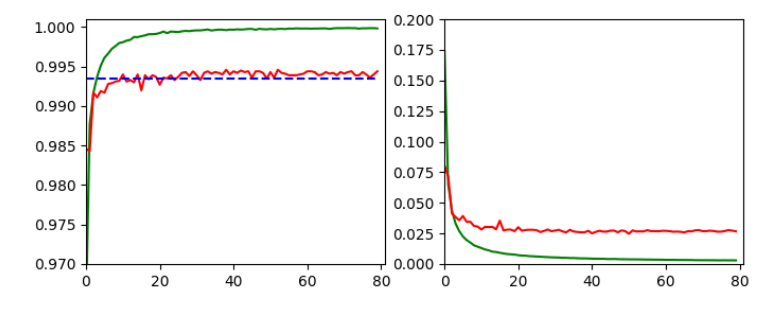and

```Epoch 80/80
933/938 [============================>.] - ETA: 0s - loss: 0.0030 - accuracy: 0.9998
present lr:  1.31509732e-05
present iteration: 75040
938/938 [==============================] - 4s 5ms/step - loss: 0.0030 - accuracy: 0.9998 - val_loss: 0.0267 - val_accuracy: 0.9944
```

# Tests and first impressions of the convolutional layer output

Ok, let us test the code to plot the maps’ output. For the input data

```# layer - pick one of the names which you defined for your model
layer_name = "Conv2D_1"

# choose a image_set and an img number
img_set = "test_imgs"
num_img = 19
```

we get the following results: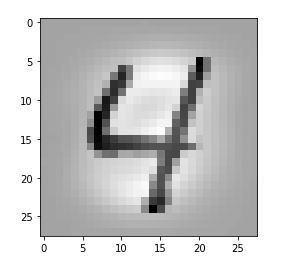Layer “Conv2D_1”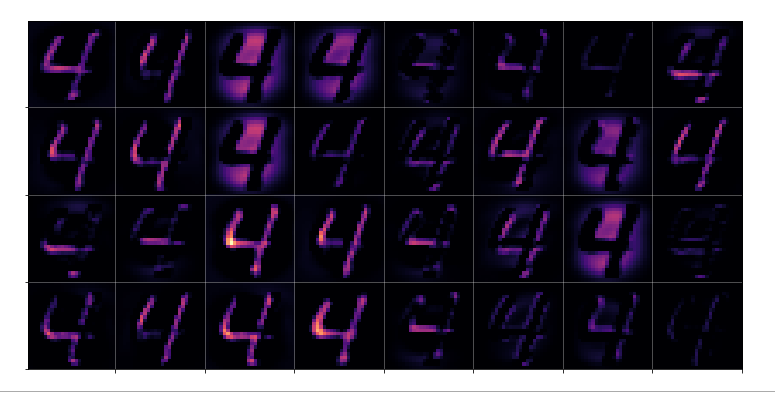Layer “Conv2D_2”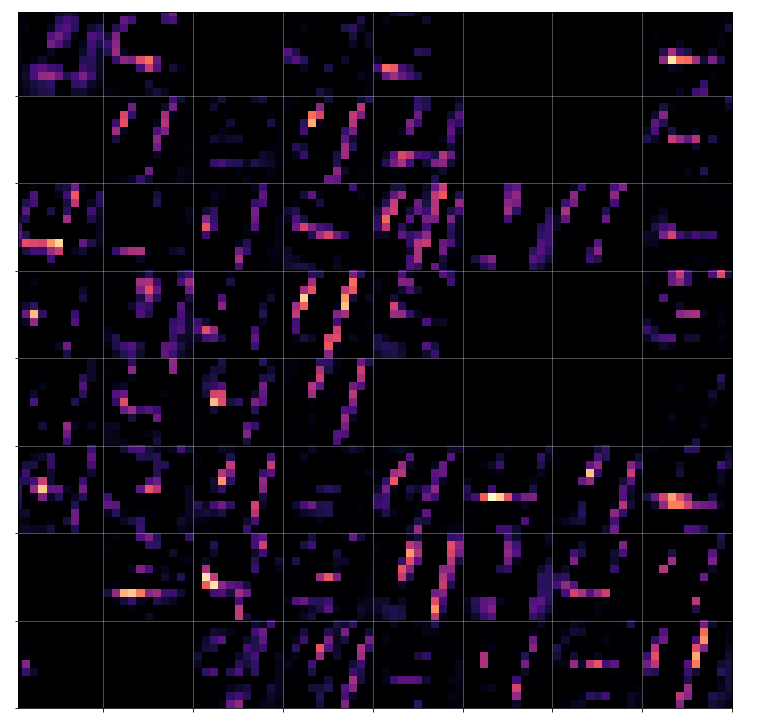Layer “Conv2D_3”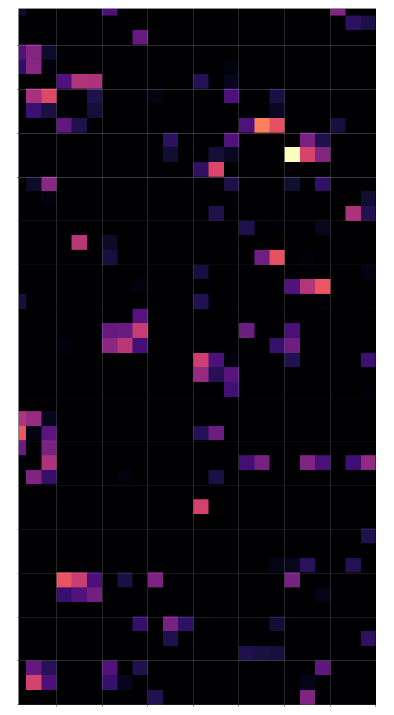# Conclusion

Keras’ flexibility regarding model definitions allows for the definition of new models based on parts of the original CNN. The output layer of these new models can be set to any of the convolutional or pooling layers. With predictions for an input image we can extract the activation results of all maps of a layer. These data can be visualized in form of a grid that shows the reaction of a layer to the input image. A first test shows that the representations of the input get more and more abstract with higher convolutional layers.

In the next article

A simple CNN for the MNIST dataset – V – about the difference of activation patterns and features

we shall have a closer look of what these abstractions may mean for the classification of certain digit images.